# Word Embeddings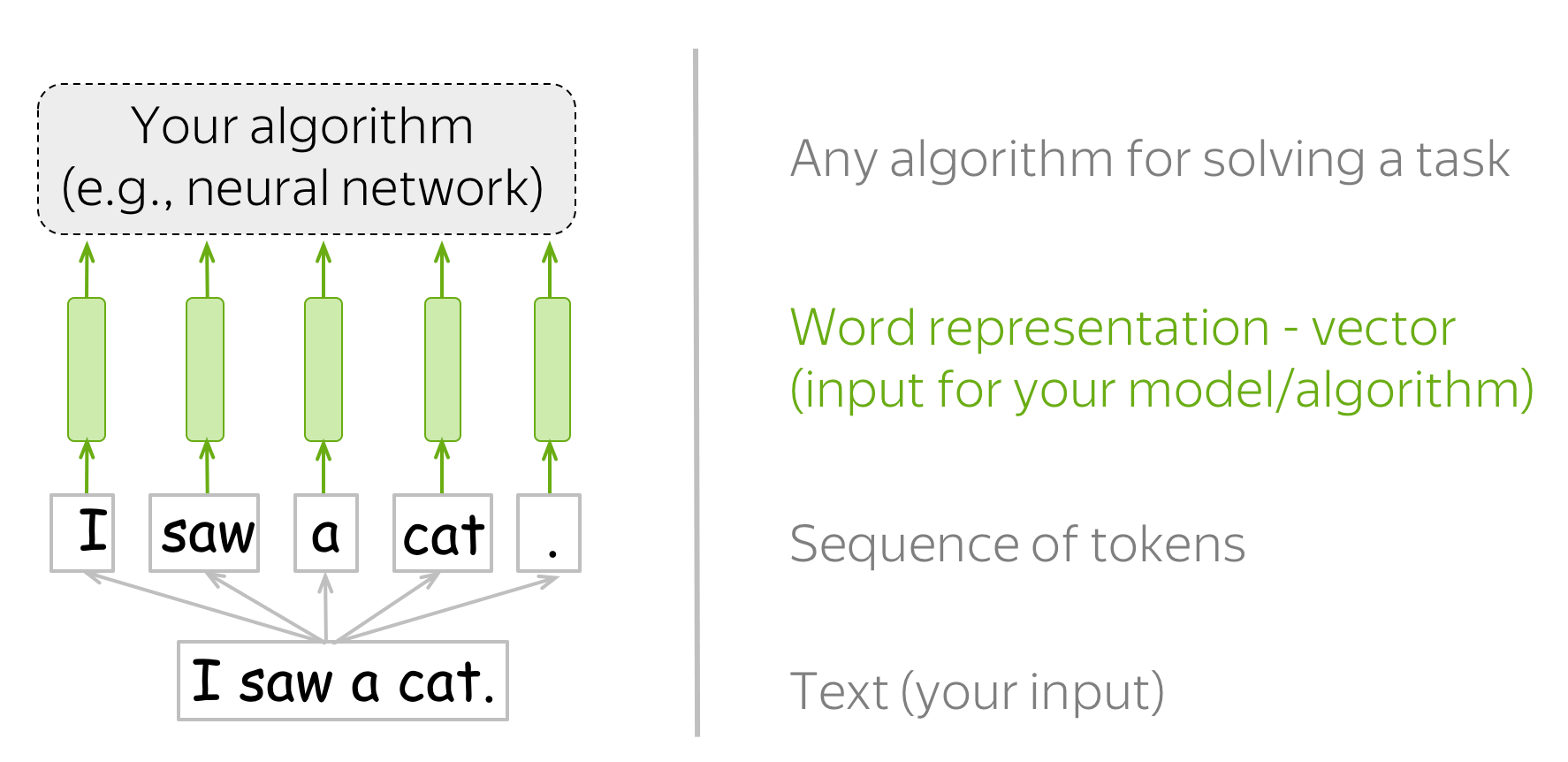The way machine learning models "see" data is different from how we (humans) do. For example, we can easily understand the text "I saw a cat", but our models can not - they need vectors of features. Such vectors, or word embeddings, are representations of words which can be fed into your model.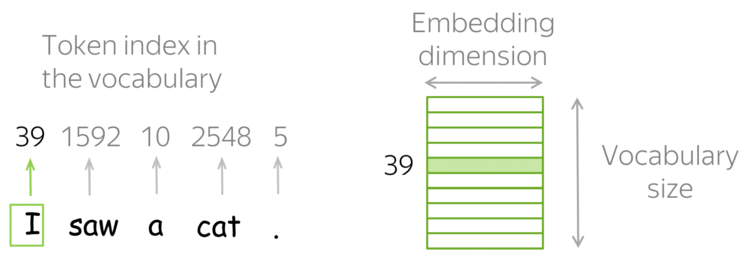#### How it works: Look-up Table (Vocabulary)

In practice, you have a vocabulary of allowed words; you choose this vocabulary in advance. For each vocabulary word, a look-up table contains its embedding. This embedding can be found using the word index in the vocabulary (i.e., you to look up the embedding in the table using word index).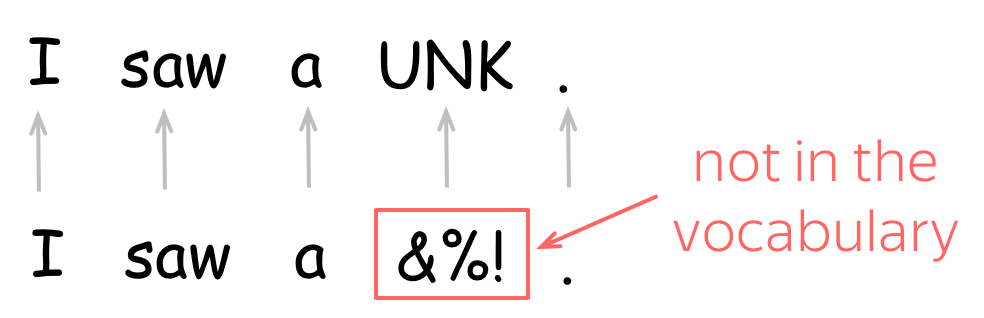To account for unknown words (the ones which are not in the vocabulary), usually a vocabulary contains a special token UNK. Alternatively, unknown tokens can be ignored or assigned a zero vector.

## Represent as Discrete Symbols: One-hot Vectors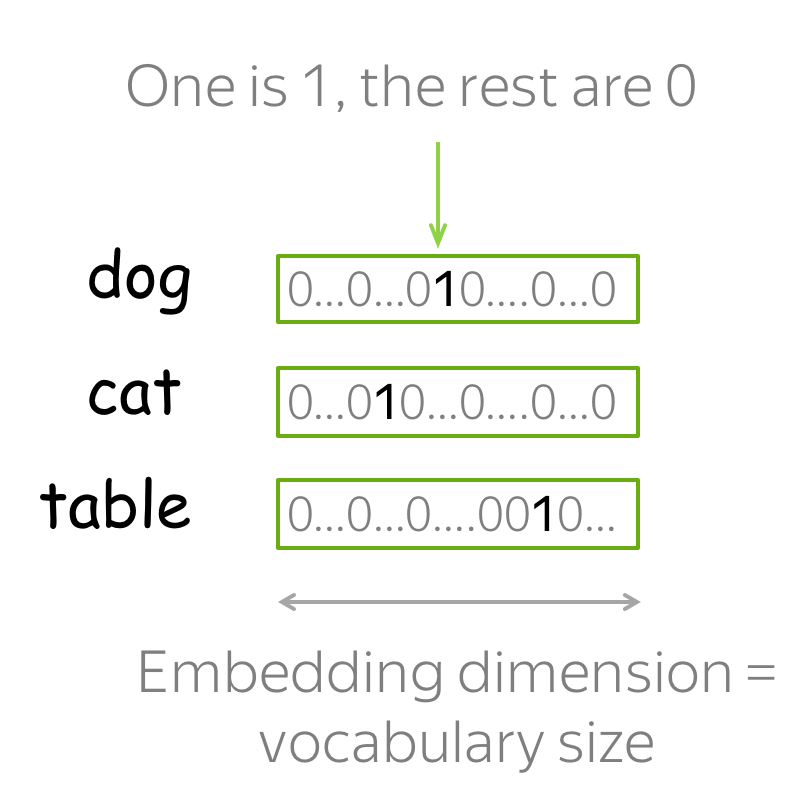The easiest you can do is to represent words as one-hot vectors: for the i-th word in the vocabulary, the vector has 1 on the i-th dimension and 0 on the rest. In Machine Learning, this is the most simple way to represent categorical features.

You probably can guess why one-hot vectors are not the best way to represent words. One of the problems is that for large vocabularies, these vectors will be very long: vector dimensionality is equal to the vocabulary size. This is undesirable in practice, but this problem is not the most crucial one.

What is really important, is that these vectors know nothing about the words they represent. For example, one-hot vectors "think" that cat is as close to dog as it is to table! We can say that one-hot vectors do not capture meaning.

But how do we know what is meaning?

## Distributional Semantics

To capture meaning of words in their vectors, we first need to define the notion of meaning that can be used in practice. For this, let us try to understand how we, humans, get to know which words have similar meaning.How to: go over the slides at your pace. Try to notice how your brain works.

Lena: The example is from Jacob Eisenstein's NLP notes; the tezgüino example originally appeared in Lin, 1998.Once you saw how the unknown word used in different contexts, you were able to understand it's meaning. How did you do this?

The hypothesis is that your brain searched for other words that can be used in the same contexts, found some (e.g., wine), and made a conclusion that tezgüino has meaning similar to those other words. This is the distributional hypothesis:

Words which frequently appear in similar contexts have similar meaning.

Lena: Often you can find it formulated as "You shall know a word by the company it keeps" with the reference to J. R. Firth in 1957, but actually there were a lot more people responsible, and much earlier. For example, Harris, 1954.

This is an extremely valuable idea: it can be used in practice to make word vectors capture their meaning. According to the distributional hypothesis, "to capture meaning" and "to capture contexts" are inherently the same. Therefore, all we need to do is to put information about word contexts into word representation.

Main idea: We need to put information about word contexts into word representation.

All we'll be doing at this lecture is looking at different ways to do this.

# Count-Based Methods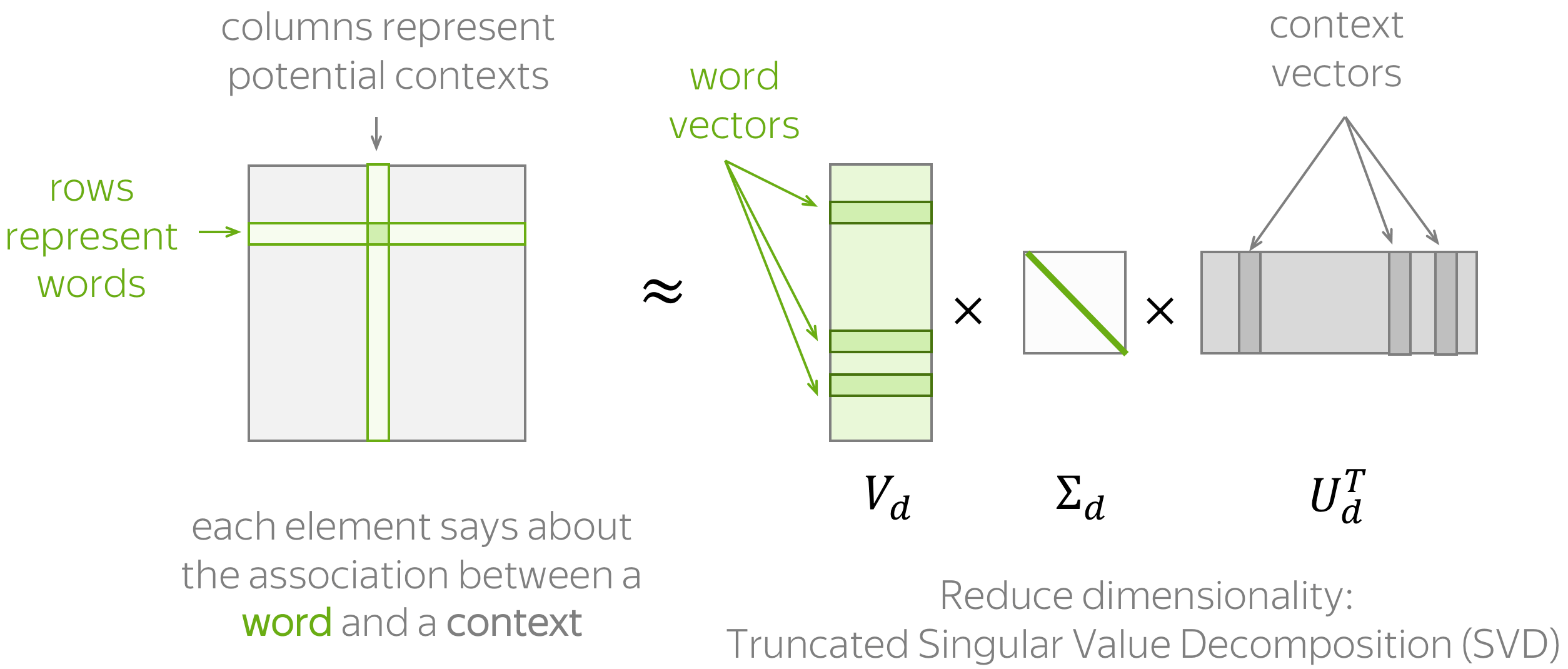Let's remember our main idea:

Main idea: We have to put information about contexts into word vectors.

Count-based methods take this idea quite literally:

How: Put this information manually based on global corpus statistics.

The general procedure is illustrated above and consists of the two steps: (1) construct a word-context matrix, (2) reduce its dimensionality. There are two reasons to reduce dimensionality. First, a raw matrix is very large. Second, since a lot of words appear in only a few of possible contexts, this matrix potentially has a lot of uninformative elements (e.g., zeros).

To estimate similarity between words/contexts, usually you need to evaluate the dot-product of normalized word/context vectors (i.e., cosine similarity).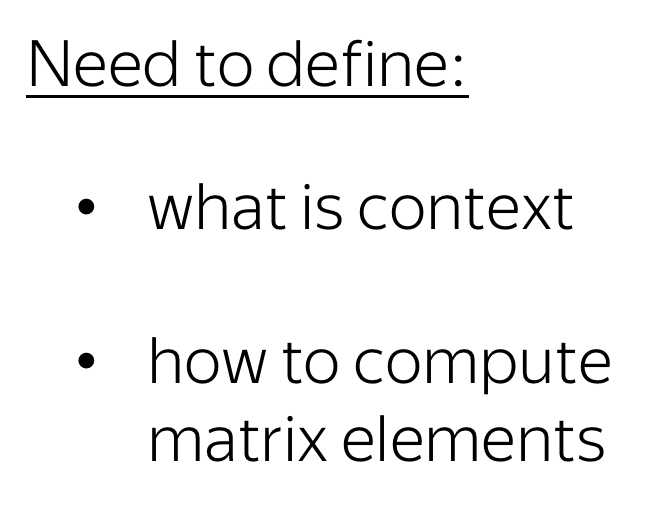To define a count-based method, we need to define two things:

• possible contexts (including what does it mean that a word appears in a context),
• the notion of association, i.e., formulas for computing matrix elements.

Below we provide a couple of popular ways of doing this.

## Simple: Co-Occurence Counts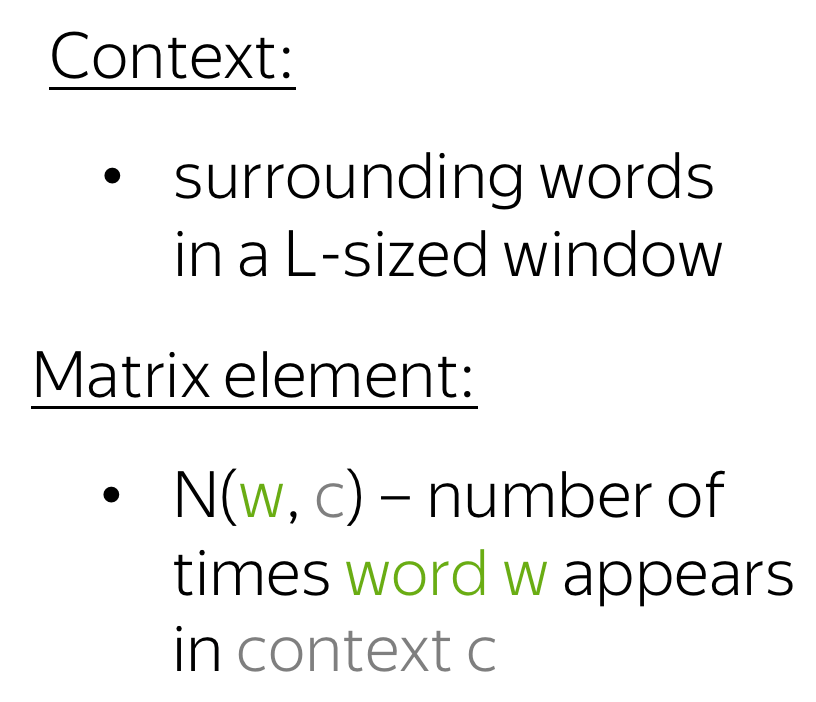The simplest approach is to define contexts as each word in an L-sized window. Matrix element for a word-context pair (w, c) is the number of times w appears in context c. This is the very basic (and very, very old) method for obtaining embeddings.The (once) famous HAL model (1996) is also a modification of this approach. Learn more from this exercise in the Research Thinking section.

## Positive Pointwise Mutual Information (PPMI)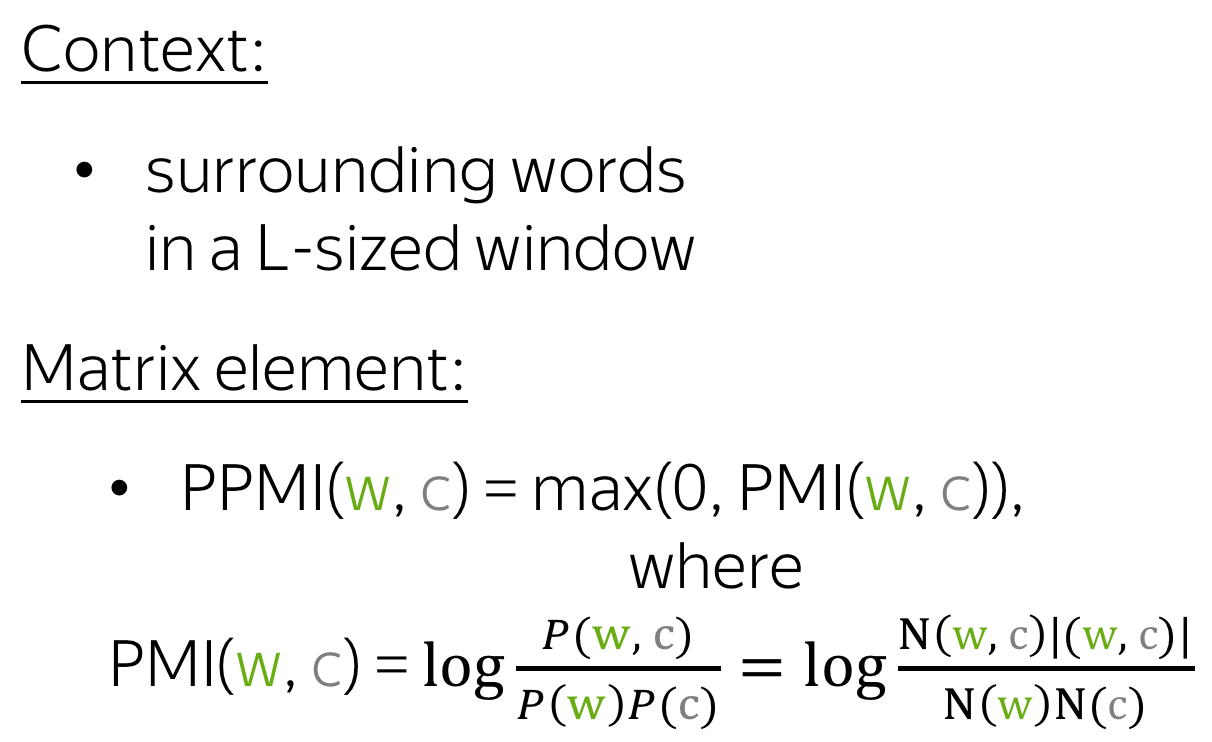Here contexts are defined as before, but the measure of the association between word and context is more clever: positive PMI (or PPMI for short). PPMI measure is widely regarded as state-of-the-art for pre-neural distributional-similarity models.

Important: relation to neural models!
Turns out, some of the neural methods we will consider (Word2Vec) were shown to implicitly approximate the factorization of a (shifted) PMI matrix. Stay tuned!

## Latent Semantic Analysis (LSA): Understanding Documents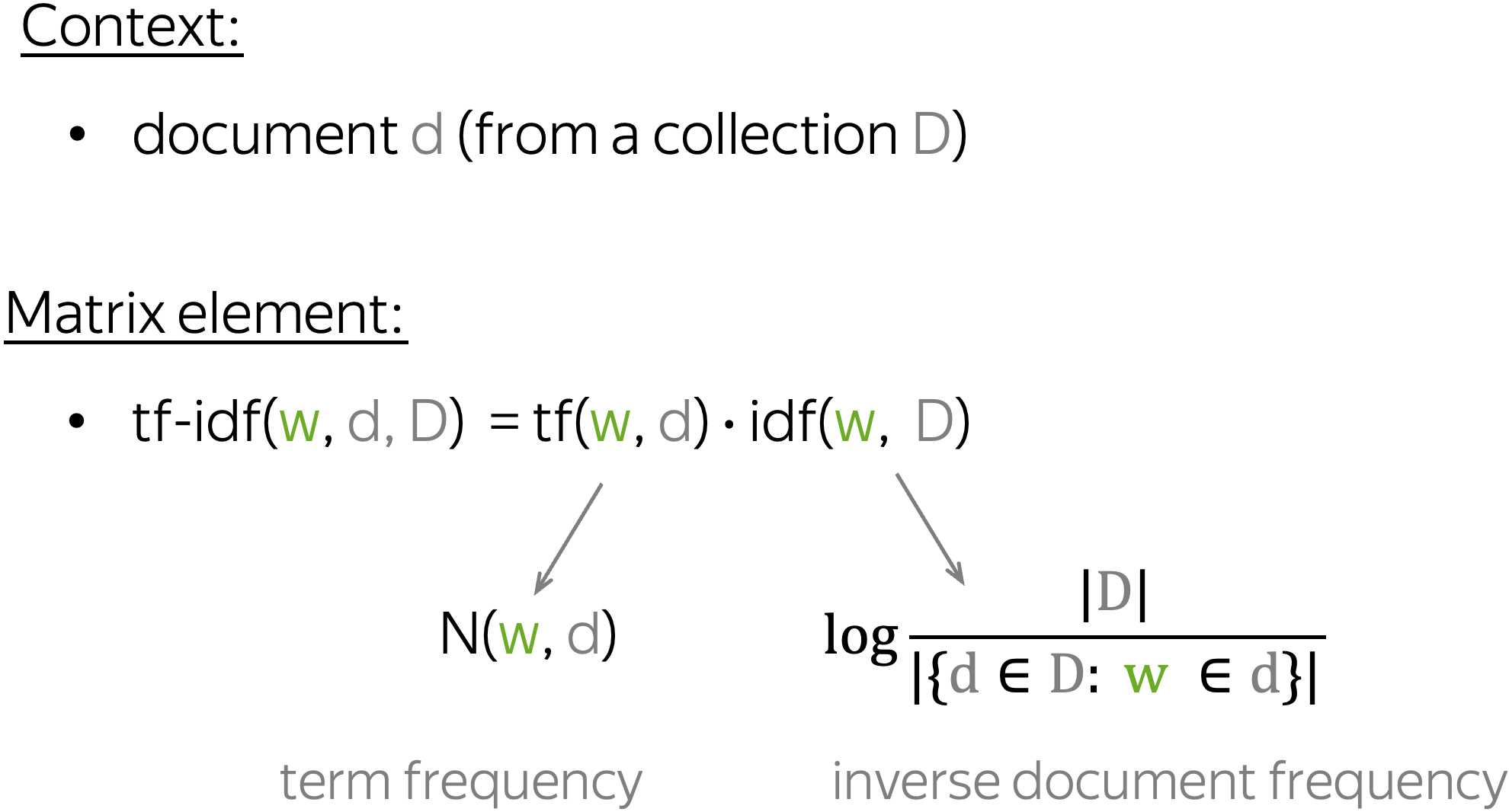Latent Semantic Analysis (LSA) analyzes a collection of documents. While in the previous approaches contexts served only to get word vectors and were thrown away afterward, here we are also interested in context, or, in this case, document vectors. LSA is one of the simplest topic models: cosine similarity between document vectors can be used to measure similarity between documents.

The term "LSA" sometimes refers to a more general approach of applying SVD to a term-document matrix where the term-document elements can be computed in different ways (e.g., simple co-occurrence, tf-idf, or some other weighting).

Animation alert! LSA wikipedia page has a nice animation of the topic detection process in a document-word matrix - take a look!

# Word2Vec: a Prediction-Based Method

Let us remember our main idea again:

Main idea: We have to put information about contexts into word vectors.

While count-based methods took this idea quite literally, Word2Vec uses it in a different manner:

How: Learn word vectors by teaching them to predict contexts.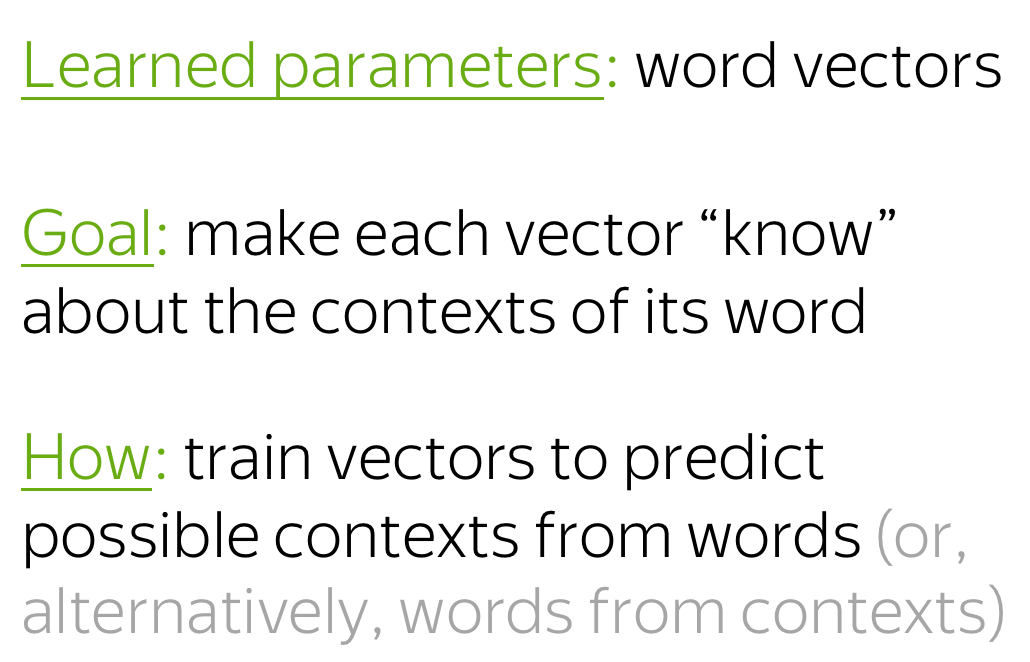Word2Vec is a model whose parameters are word vectors. These parameters are optimized iteratively for a certain objective. The objective forces word vectors to "know" contexts a word can appear in: the vectors are trained to predict possible contexts of the corresponding words. As you remember from the distributional hypothesis, if vectors "know" about contexts, they "know" word meaning.

Word2Vec is an iterative method. Its main idea is as follows:

• take a huge text corpus;
• go over the text with a sliding window, moving one word at a time. At each step, there is a central word and context words (other words in this window);
• for the central word, compute probabilities of context words;
• adjust the vectors to increase these probabilities.How to: go over the illustration to understand the main idea.

Lena: Visualization idea is from the Stanford CS224n course.## Objective Function: Negative Log-Likelihood

For each position $$t =1, \dots, T$$ in a text corpus, Word2Vec predicts context words within a m-sized window given the central word $$\color{#88bd33}{w_t}$$: $\color{#88bd33}{\mbox{Likelihood}} \color{black}= L(\theta)= \prod\limits_{t=1}^T\prod\limits_{-m\le j \le m, j\neq 0}P(\color{#888}{w_{t+j}}|\color{#88bd33}{w_t}\color{black}, \theta),$ where $$\theta$$ are all variables to be optimized. The objective function (aka loss function or cost function) $$J(\theta)$$ is the average negative log-likelihood: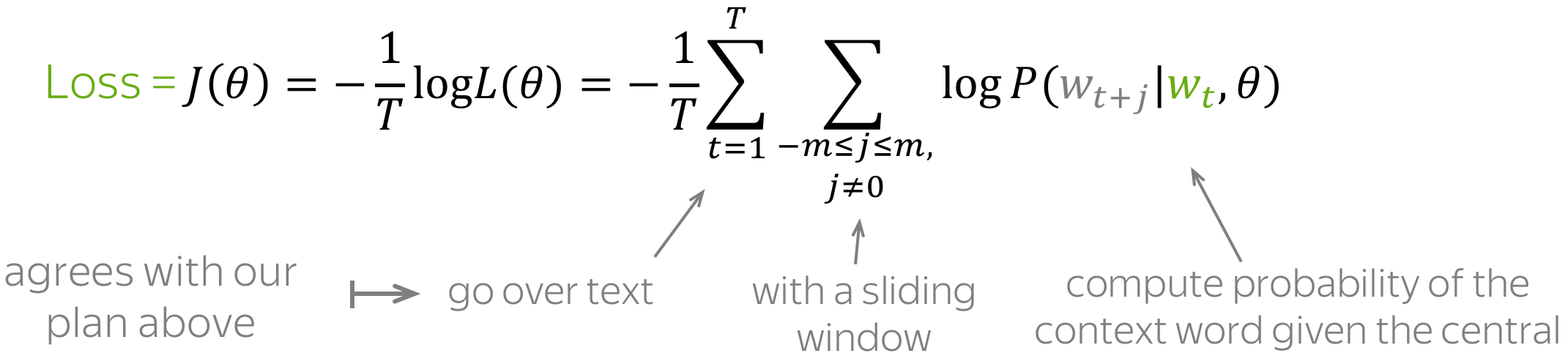Note how well the loss agrees with our plan main above: go over text with a sliding window and compute probabilities. Now let's find out how to compute these probabilities.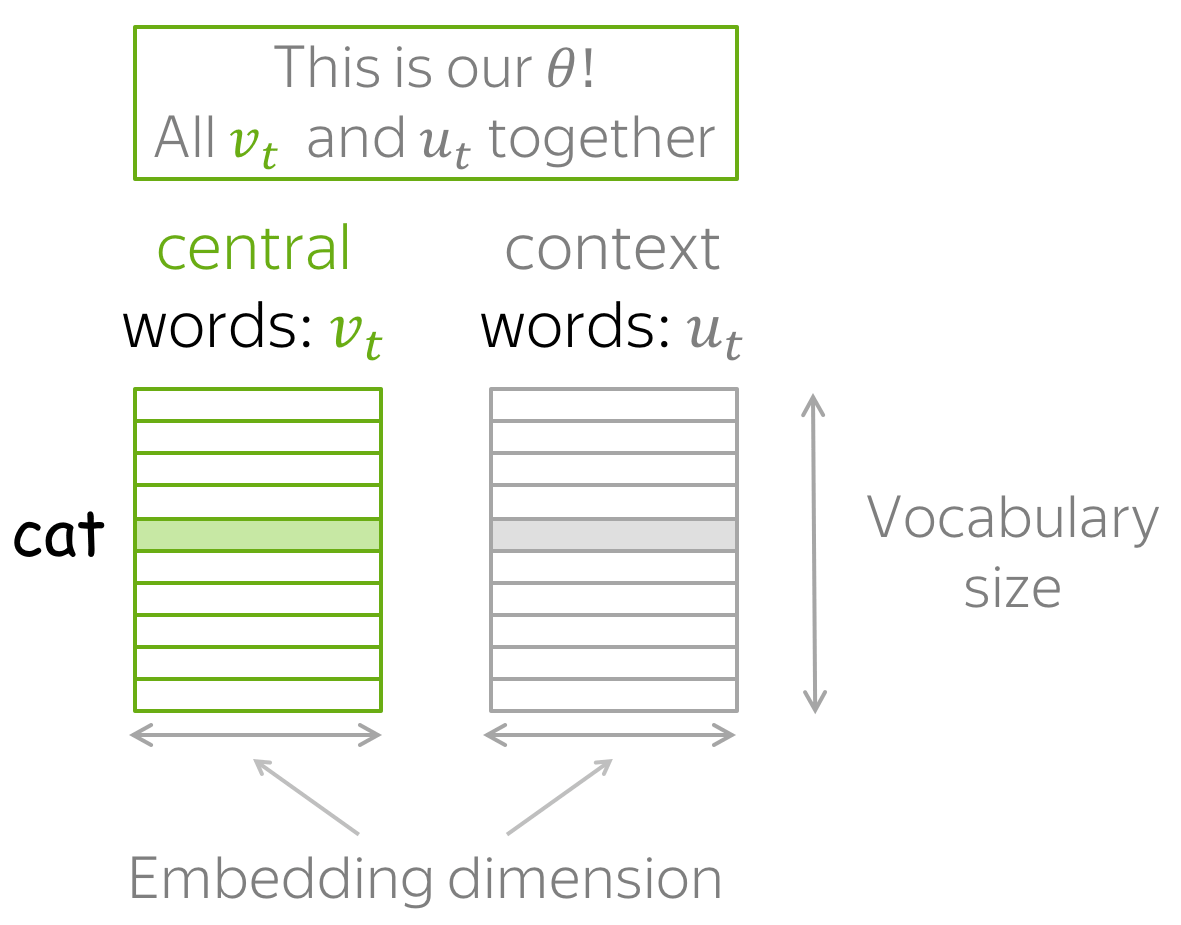### How to calculate $$P(\color{#888}{w_{t+j}}\color{black}|\color{#88bd33}{w_t}\color{black}, \theta)$$?

For each word $$w$$ we will have two vectors:

• $$\color{#88bd33}{v_w}$$ when it is a central word;
• $$\color{#888}{u_w}$$ when it is a context word.

(Once the vectors are trained, usually we throw away context vectors and use only word vectors.)

Then for the central word $$\color{#88bd33}{c}$$ (c - central) and the context word $$\color{#888}{o}$$ (o - outside word) probability of the context word is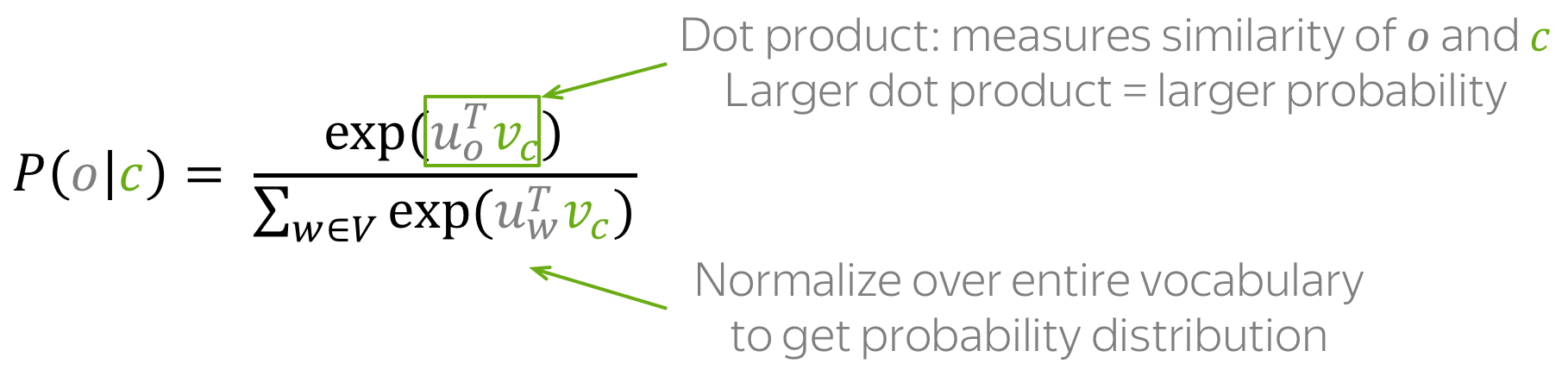Note: this is the softmax function! (click for the details)

The function above is an example of the softmax function: $softmax(x_i)=\frac{\exp(x_i)}{\sum\limits_{j=i}^n\exp(x_j)}.$ Softmax maps arbitrary values $$x_i$$ to a probability distribution $$p_i$$:

• "max" because the largest $$x_i$$ will have the largest probability $$p_i$$;
• "soft" because all probabilities are non-zero.

You will deal with this function quite a lot over the NLP course (and in Deep Learning in general).How to: go over the illustration. Note that for central words and context words, different vectors are used. For example, first the word a is central and we use $$\color{#88bd33}{v_a}$$, but when it becomes context, we use $$\color{#888}{u_a}$$ instead.## How to train: by Gradient Descent, One Word at a Time

Let us recall that our parameters $$\theta$$ are vectors $$\color{#88bd33}{v_w}$$ and $$\color{#888}{u_w}$$ for all words in the vocabulary. These vectors are learned by optimizing the training objective via gradient descent (with some learning rate $$\alpha$$): $\theta^{new} = \theta^{old} - \alpha \nabla_{\theta} J(\theta).$

### One word at a time

We make these updates one at a time: each update is for a single pair of a center word and one of its context words. Look again at the loss function: $\color{#88bd33}{\mbox{Loss}}\color{black} =J(\theta)= -\frac{1}{T}\log L(\theta)= -\frac{1}{T}\sum\limits_{t=1}^T \sum\limits_{-m\le j \le m, j\neq 0}\log P(\color{#888}{w_{t+j}}\color{black}|\color{#88bd33}{w_t}\color{black}, \theta)= \frac{1}{T} \sum\limits_{t=1}^T \sum\limits_{-m\le j \le m, j\neq 0} J_{t,j}(\theta).$ For the center word $$\color{#88bd33}{w_t}$$, the loss contains a distinct term $$J_{t,j}(\theta)=-\log P(\color{#888}{w_{t+j}}\color{black}|\color{#88bd33}{w_t}\color{black}, \theta)$$ for each of its context words $$\color{#888}{w_{t+j}}$$. Let us look in more detail at just this one term and try to understand how to make an update for this step. For example, let's imagine we have a sentencewith the central word cat, and four context words. Since we are going to look at just one step, we will pick only one of the context words; for example, let's take cute. Then the loss term for the central word cat and the context word cute is: $J_{t,j}(\theta)= -\log P(\color{#888}{cute}\color{black}|\color{#88bd33}{cat}\color{black}) = -\log \frac{\exp\color{#888}{u_{cute}^T}\color{#88bd33}{v_{cat}}}{ \sum\limits_{w\in Voc}\exp{\color{#888}{u_w^T}\color{#88bd33}{v_{cat}} }} = -\color{#888}{u_{cute}^T}\color{#88bd33}{v_{cat}}\color{black} + \log \sum\limits_{w\in Voc}\exp{\color{#888}{u_w^T}\color{#88bd33}{v_{cat}}}\color{black}{.}$

Note which parameters are present at this step:

• from vectors for central words, only $$\color{#88bd33}{v_{cat}}$$;
• from vectors for context words, all $$\color{#888}{u_w}$$ (for all words in the vocabulary).

Only these parameters will be updated at the current step.

Below is the schematic illustration of the derivations for this step.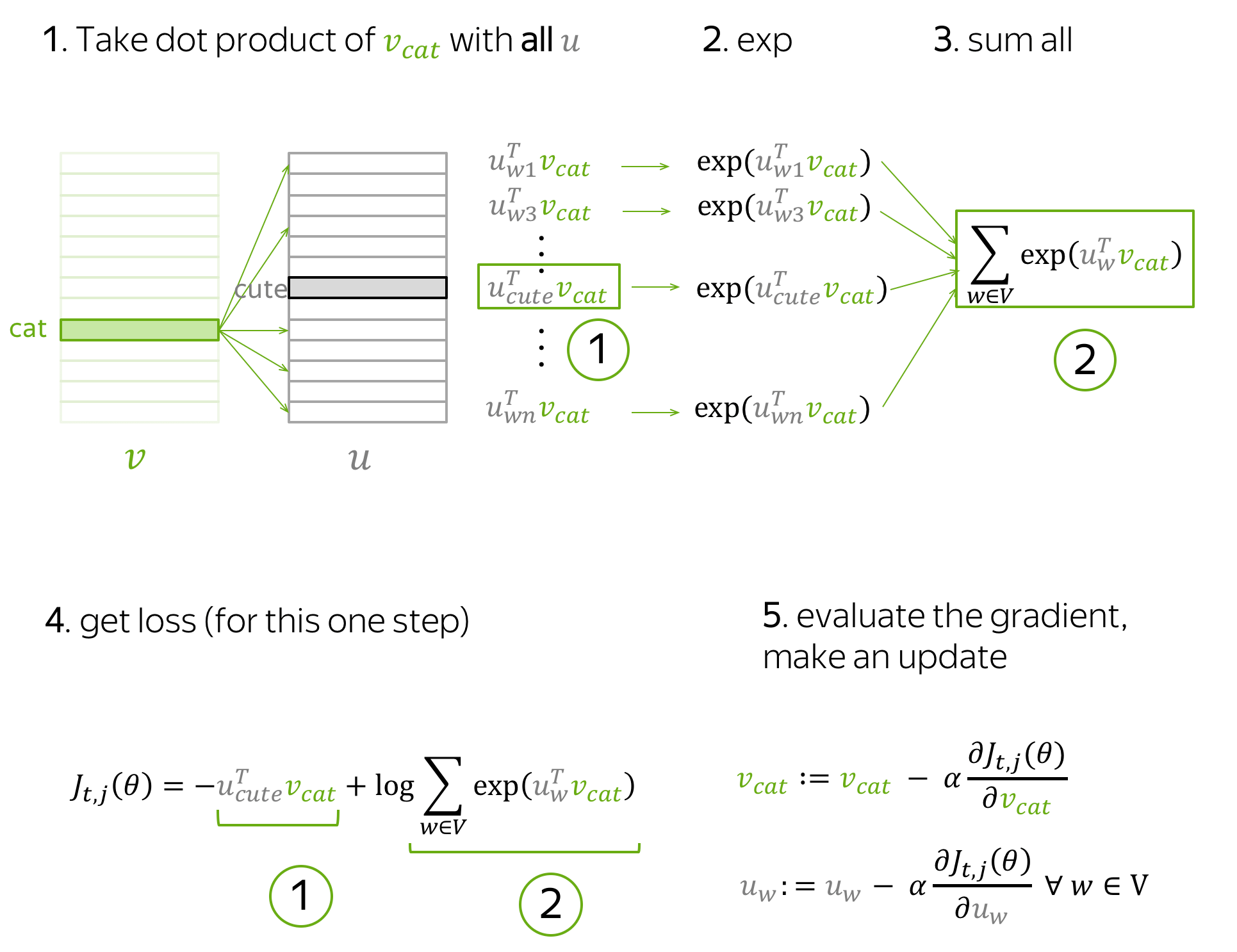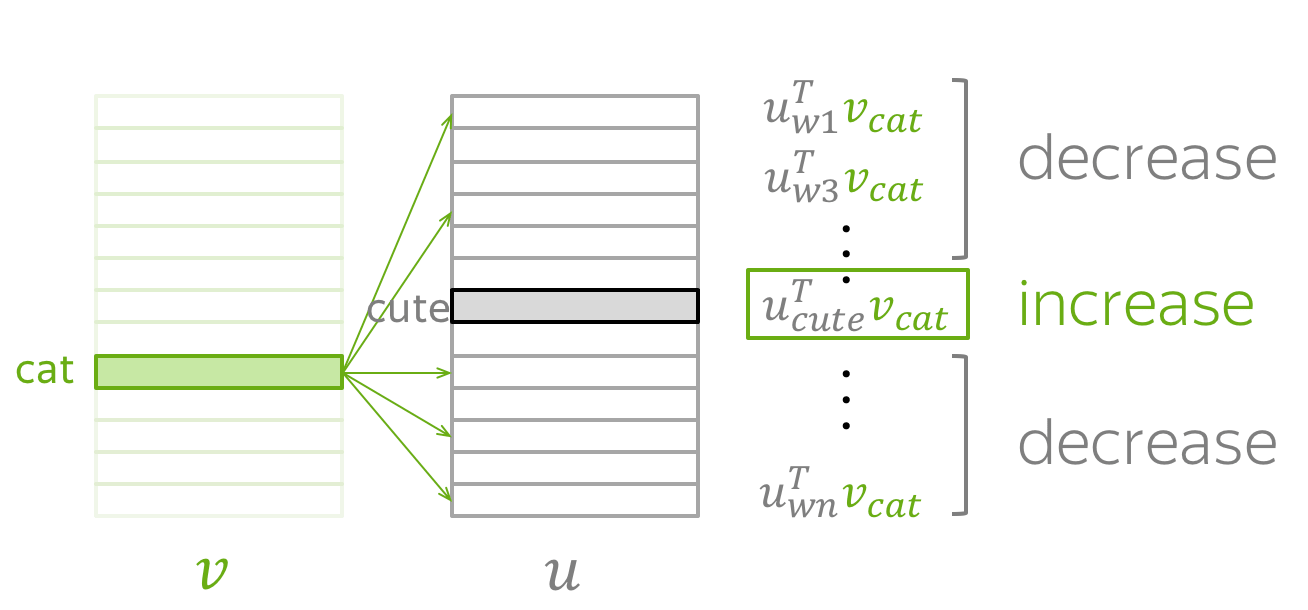By making an update to minimize $$J_{t,j}(\theta)$$, we force the parameters to increase similarity (dot product) of $$\color{#88bd33}{v_{cat}}$$ and $$\color{#888}{u_{cute}}$$ and, at the same time, to decrease similarity between $$\color{#88bd33}{v_{cat}}$$ and $$\color{#888}{u_{w}}$$ for all other words $$w$$ in the vocabulary.

This may sound a bit strange: why do we want to decrease similarity between $$\color{#88bd33}{v_{cat}}$$ and all other words, if some of them are also valid context words (e.g., grey, playing, in on our example sentence)?
But do not worry: since we make updates for each context word (and for all central words in your text), on average over all updates our vectors will learn the distribution of the possible contexts.Try to derive the gradients at the final step of the illustration above.

If you get lost, you can look at the paper Word2Vec Parameter Learning Explained.

## Faster Training: Negative Sampling

In the example above, for each pair of a central word and its context word, we had to update all vectors for context words. This is highly inefficient: for each step, the time needed to make an update is proportional to the vocabulary size.

But why do we have to consider all context vectors in the vocabulary at each step? For example, imagine that at the current step we consider context vectors not for all words, but only for the current target (cute) and several randomly chosen words. The figure shows the intuition.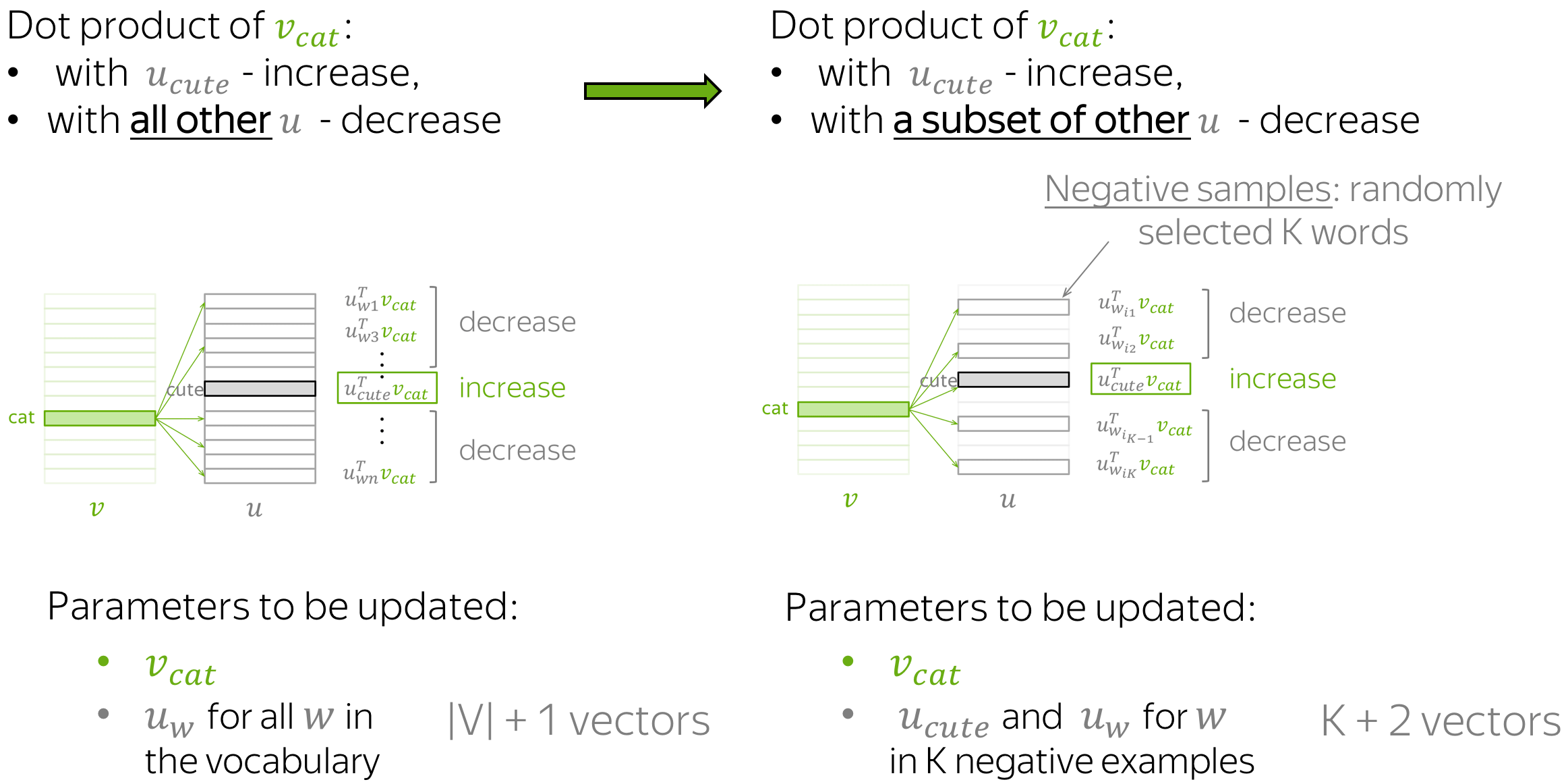As before, we are increasing similarity between $$\color{#88bd33}{v_{cat}}$$ and $$\color{#888}{u_{cute}}$$. What is different, is that now we decrease similarity between $$\color{#88bd33}{v_{cat}}$$ and context vectors not for all words, but only with a subset of K "negative" examples.

Since we have a large corpus, on average over all updates we will update each vector sufficient number of times, and the vectors will still be able to learn the relationships between words quite well.

Formally, the new loss function for this step is: $J_{t,j}(\theta)= -\log\sigma(\color{#888}{u_{cute}^T}\color{#88bd33}{v_{cat}}\color{black}) - \sum\limits_{w\in \{w_{i_1},\dots, w_{i_K}\}}\log\sigma({-\color{#888}{u_w^T}\color{#88bd33}{v_{cat}}}\color{black}),$ where $$w_{i_1},\dots, w_{i_K}$$ are the K negative examples chosen at this step and $$\sigma(x)=\frac{1}{1+e^{-x}}$$ is the sigmoid function.

Note that $$\sigma(-x)=\frac{1}{1+e^{x}}=\frac{1\cdot e^{-x}}{(1+e^{x})\cdot e^{-x}} = \frac{e^{-x}}{1+e^{-x}}= 1- \frac{1}{1+e^{x}}=1-\sigma(x)$$. Then the loss can also be written as: $J_{t,j}(\theta)= -\log\sigma(\color{#888}{u_{cute}^T}\color{#88bd33}{v_{cat}}\color{black}) - \sum\limits_{w\in \{w_{i_1},\dots, w_{i_K}\}}\log(1-\sigma({\color{#888}{u_w^T}\color{#88bd33}{v_{cat}}}\color{black})).$### The Choice of Negative Examples

Each word has only a few "true" contexts. Therefore, randomly chosen words are very likely to be "negative", i.e. not true contexts. This simple idea is used not only to train Word2Vec efficiently but also in many other applications, some of which we will see later in the course.

Word2Vec randomly samples negative examples based on the empirical distribution of words. Let $$U(w)$$ be a unigram distribution of words, i.e. $$U(w)$$ is the frequency of the word $$w$$ in the text corpus. Word2Vec modifies this distribution to sample less frequent words more often: it samples proportionally to $$U^{3/4}(w)$$.

## Word2Vec variants: Skip-Gram and CBOW

There are two Word2Vec variants: Skip-Gram and CBOW.

Skip-Gram is the model we considered so far: it predicts context words given the central word. Skip-Gram with negative sampling is the most popular approach.

CBOW (Continuous Bag-of-Words) predicts the central word from the sum of context vectors. This simple sum of word vectors is called "bag of words", which gives the name for the model.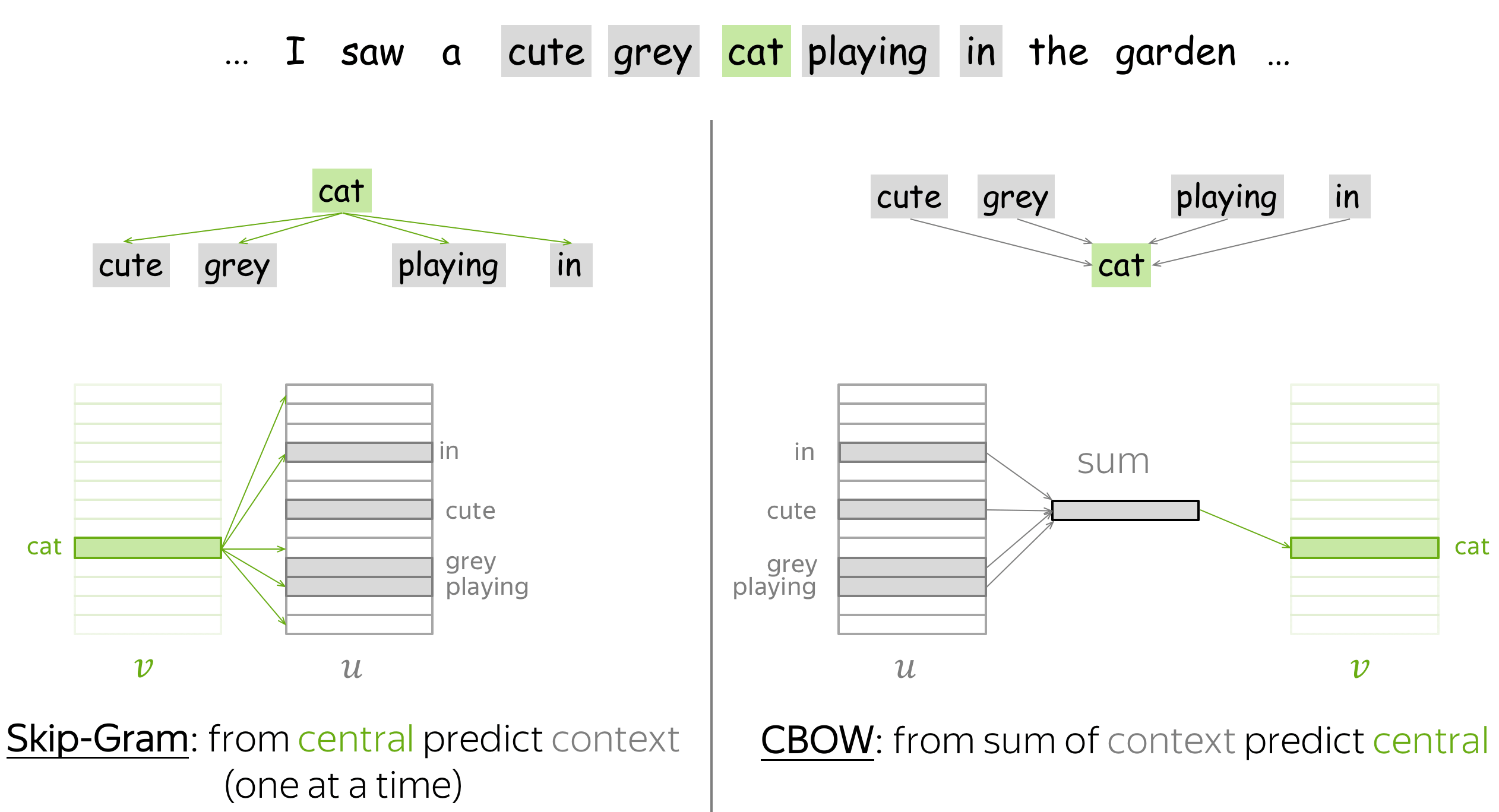How the loss function and the gradients change for the CBOW model?

If you get lost, you can again look at the paper Word2Vec Parameter Learning Explained.

The original Word2Vec papers are:

You can look into them for the details on the experiments, implementation and hyperparameters. Here we will provide some of the most important things you need to know.

### The Idea is Not New

The idea to learn word vectors (distributed representations) is not new. For example, there were attempts to learn word vectors as part of a larger network and then extract the embedding layer. (For the details on the previous methods, you can look, for example, at the summary in the original Word2Vec papers).

What was very unexpected in Word2Vec, is its ability to learn high-quality word vectors very fast on huge datasets and for large vocabularies. And of course, all the fun properties we will see in the Analysis and Interpretability section quickly made Word2Vec very famous.

### Why Two Vectors?

As you remember, in Word2Vec we train two vectors for each word: one when it is a central word and another when it is a context word. After training, context vectors are thrown away.

This is one of the tricks that made Word2Vec so simple. Look again at the loss function (for one step): $J_{t,j}(\theta)= -\color{#888}{u_{cute}^T}\color{#88bd33}{v_{cat}}\color{black} - \log \sum\limits_{w\in V}\exp{\color{#888}{u_w^T}\color{#88bd33}{v_{cat}}}\color{black}{.}$ When central and context words have different vectors, both the first term and dot products inside the exponents are linear with respect to the parameters (the same for the negative training objective). Therefore, the gradients are easy to compute.Repeat the derivations (loss and the gradients) for the case with one vector for each word ($$\forall w \ in \ V, \color{#88bd33}{v_{w}}\color{black}{ = }\color{#888}{u_{w}}$$ ).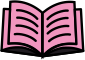While the standard practice is to throw away context vectors, it was shown that averaging word and context vectors may be more beneficial. More details are here.

### Better trainingThere's one more trick: learn more from this exercise in the Research Thinking section.

### Relation to PMI Matrix FactorizationWord2Vec SGNS (Skip-Gram with Negative Sampling) implicitly approximates the factorization of a (shifted) PMI matrix. Learn more here.

### The Effect of Window Size

The size of the sliding window has a strong effect on the resulting vector similarities. For example, this paper notes that larger windows tend to produce more topical similarities (i.e. dog, bark and leash will be grouped together, as well as walked, run and walking), while smaller windows tend to produce more functional and syntactic similarities (i.e. Poodle, Pitbull, Rottweiler, or walking, running, approaching).

### (Somewhat) Standard Hyperparameters

As always, the choice of hyperparameters usually depends on the task at hand; you can look at the original papers for more details.

Somewhat standard setting is:
• Model: Skip-Gram with negative sampling;
• Number of negative examples: for smaller datasets, 15-20; for huge datasets (which are usually used) it can be 2-5.
• Embedding dimensionality: frequently used value is 300, but other variants (e.g., 100 or 50) are also possible. For theoretical explanation of the optimal dimensionality, take a look at the Related Papers section.
• Sliding window (context) size: 5-10.

# GloVe: Global Vectors for Word Representation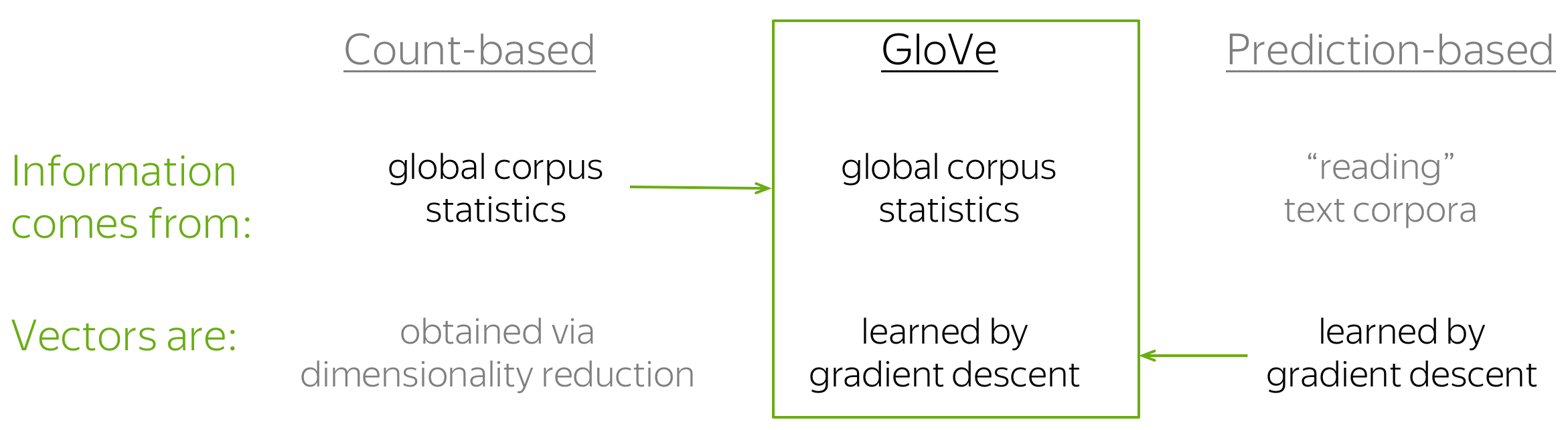The GloVe model is a combination of count-based methods and prediction methods (e.g., Word2Vec). Model name, GloVe, stands for "Global Vectors", which reflects its idea: the method uses global information from corpus to learn vectors.

As we saw earlier, the simplest count-based method uses co-occurrence counts to measure the association between word w and context c: N(w, c). GloVe also uses these counts to construct the loss function: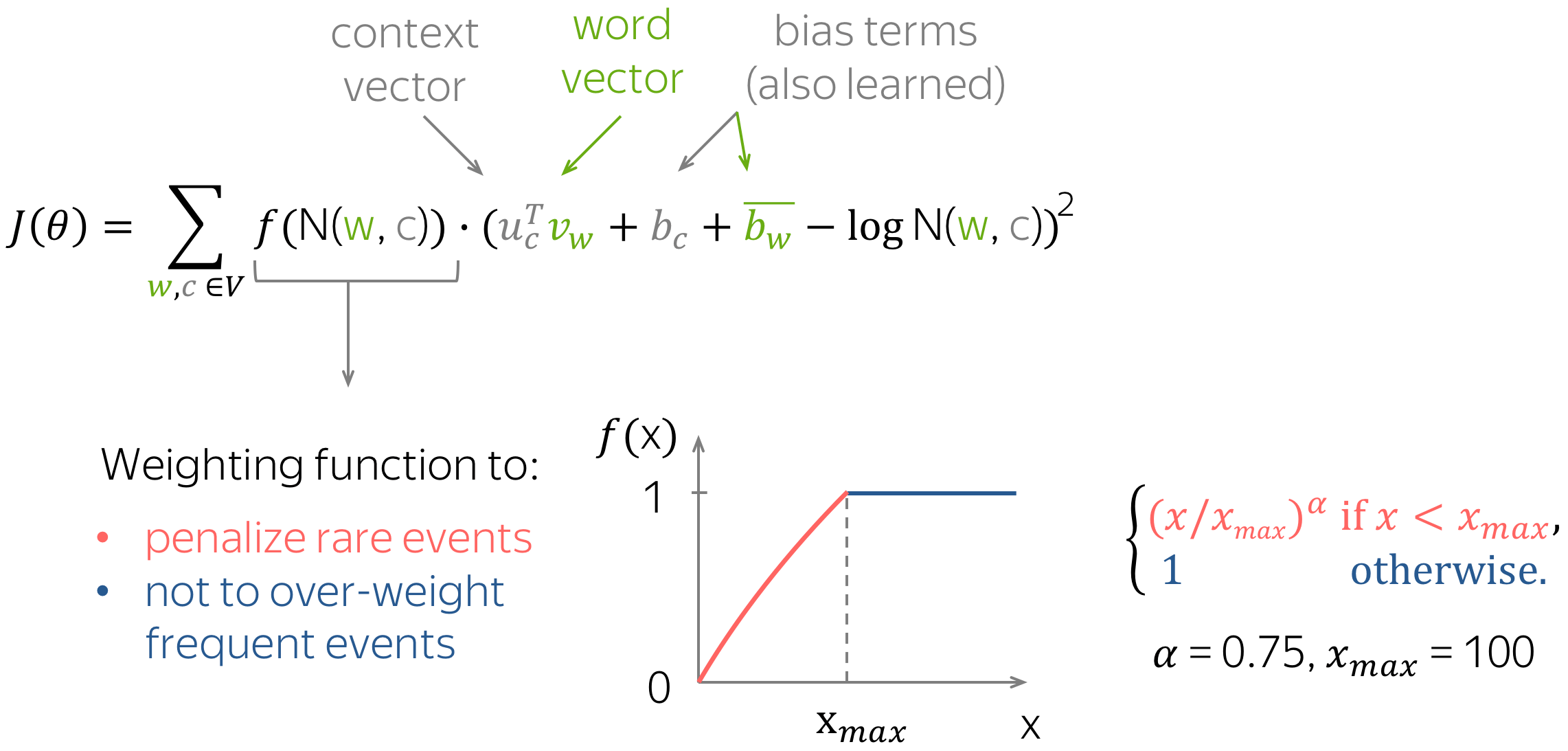Similar to Word2Vec, we also have different vectors for central and context words - these are our parameters. Additionally, the method has a scalar bias term for each word vector.

What is especially interesting, is the way GloVe controls the influence of rare and frequent words: loss for each pair (w, c) is weighted in a way that

• rare events are penalized,
• very frequent events are not over-weighted.

Lena: The loss function looks reasonable as it is, but the original GloVe paper has very nice motivation leading to the above formula. I will not provide it here (I have to finish the lecture at some point, right?..), but you can read it yourself - it's really, really nice!

# Evaluation of Word Embeddings

How can we understand that one method for getting word embeddings is better than another? There are two types of evaluation (not only for word embeddings): intrinsic and extrinsic.

### Intrinsic Evaluation: Based on Internal Properties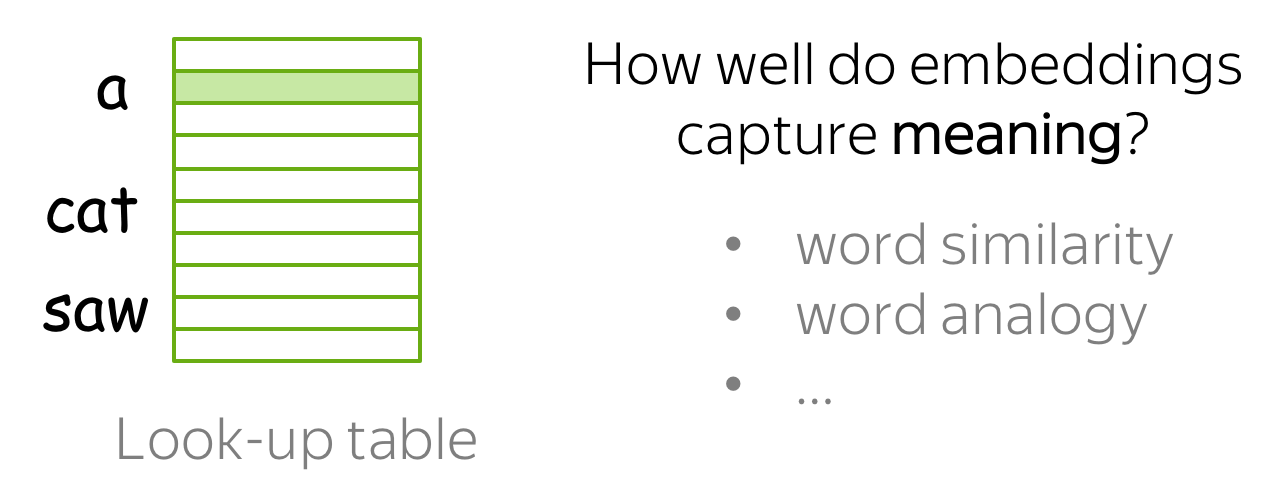This type of evaluation looks at the internal properties of embeddings, i.e. how well they capture meaning. Specifically, in the Analysis and Interpretability section, we will discuss in detail how we can evaluate embeddings on word similarity and word analogy tasks.

### Extrinsic Evaluation: On a Real Task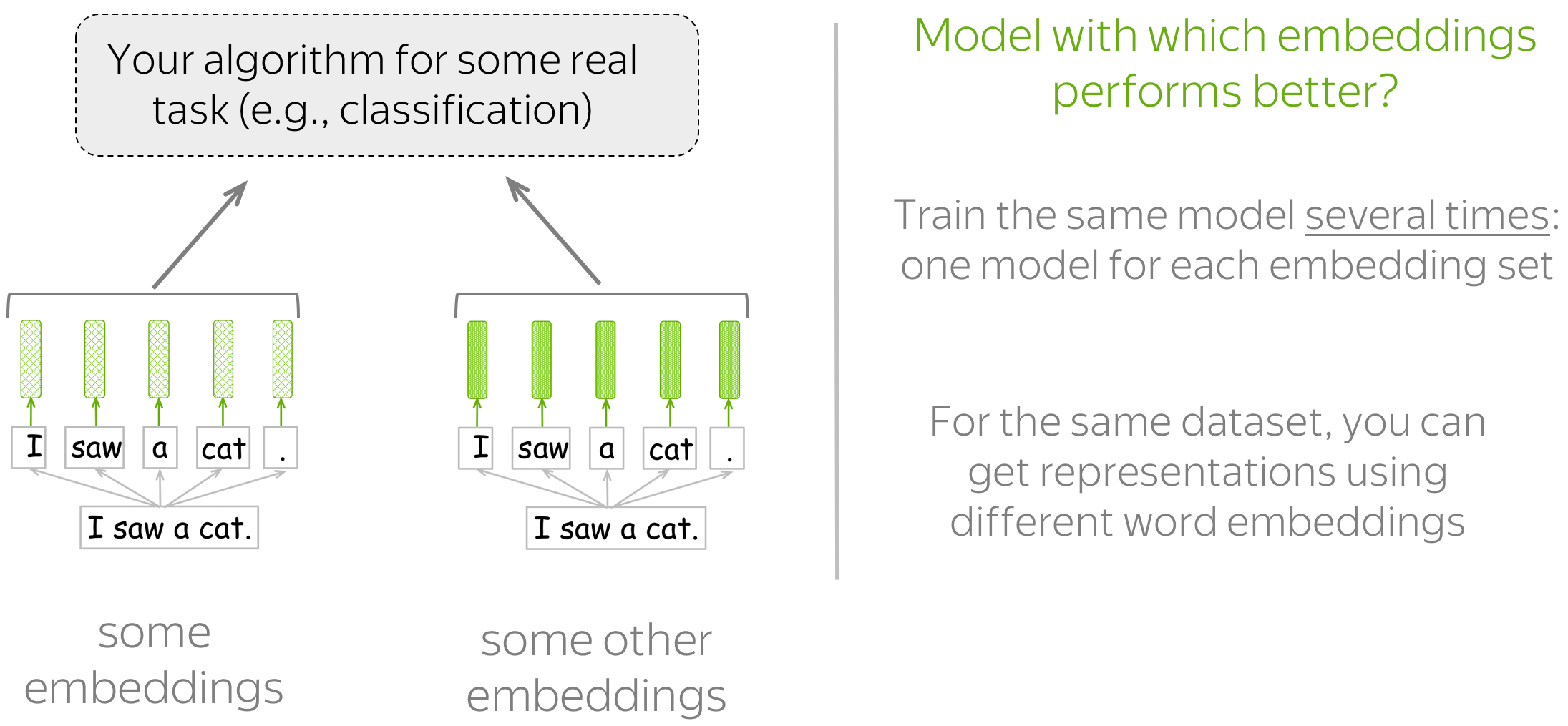This type of evaluation tells which embeddings are better for the task you really care about (e.g., text classification, coreference resolution, etc.).

In this setting, you have to train the model/algorithm for the real task several times: one model for each of the embeddings you want to evaluate. Then, look at the quality of these models to decide which embeddings are better.

### How to Choose?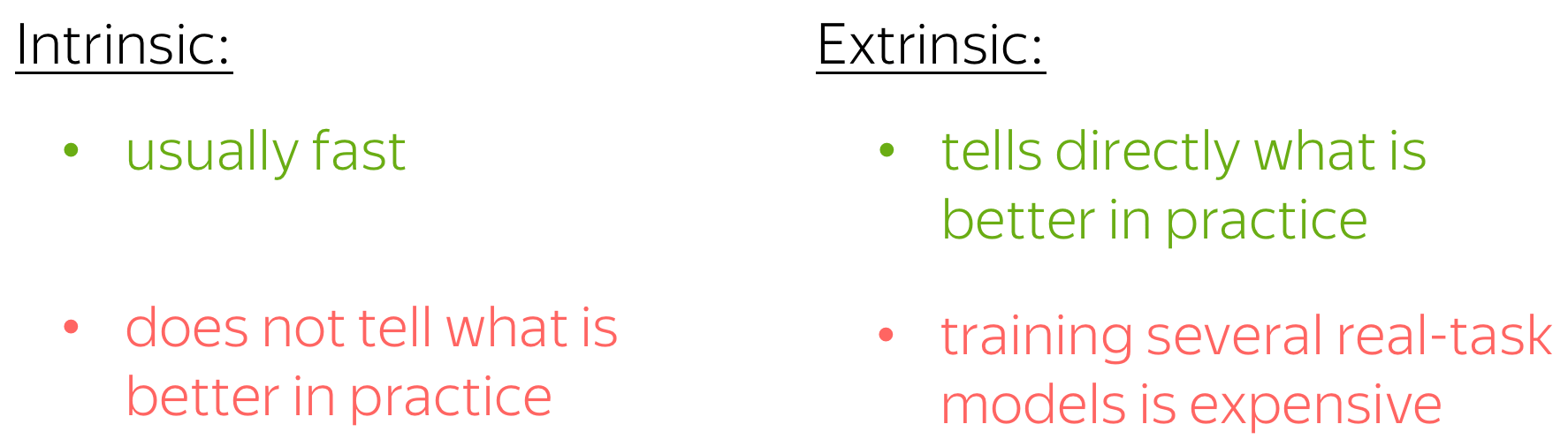One thing you have to get used to is that there is no perfect solution and no right answer for all situations: it always depends on many things.

Regarding evaluation, you usually care about quality of the task you want to solve. Therefore, you are likely to be more interested in extrinsic evaluation. However, real-task models usually require a lot of time and resources to train, and training several of them may be too expensive.

In the end, this is your call to make :)# Analysis and Interpretability

Lena: For word embeddings, most of the content of this part is usually considered as evaluation (intrinsic evaluation). However, since looking at what a model learned (beyond task-specific metrics) is the kind of thing people usually do for analysis, I believe it can be presented here, in the analysis section.

## Take a Walk Through Space... Semantic Space!

Semantic spaces aim to create representations of natural language that capture meaning. We can say that (good) word embeddings form semantic space and will refer to a set of word vectors in a multi-dimensional space as "semantic space".

Below is shown semantic space formed by GloVe vectors trained on twitter data (taken from gensim). Vectors were projected to two-dimensional space using t-SNE; these are only the top-3k most frequent words.How to: Walk through semantic space and try to find:

• language clusters: Spanish, Arabic, Russian, English. Can you find more languages?
• clusters for: food, family, names, geographical locations. What else can you find?## Nearest Neighbors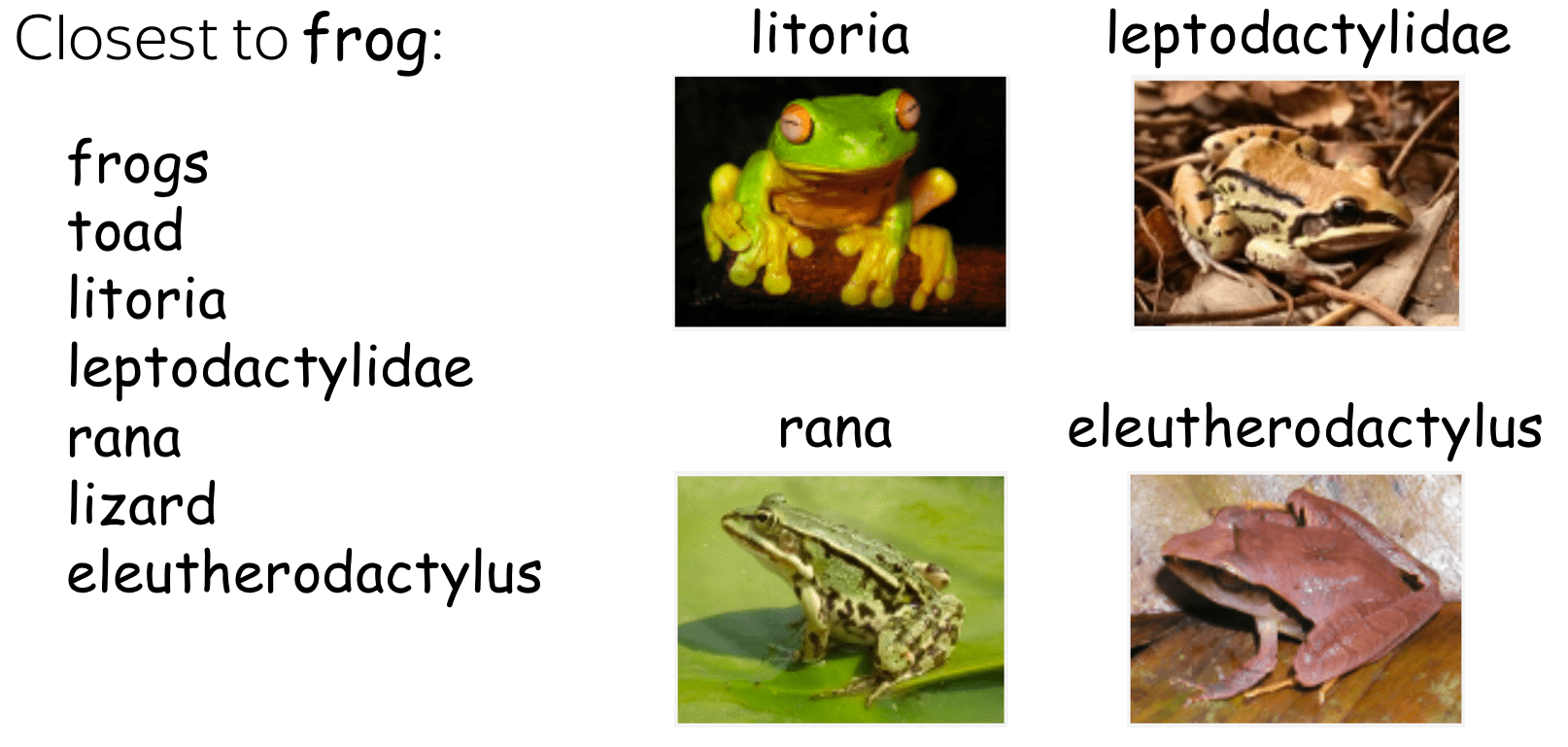The example is from the GloVe project page.

During your walk through semantic space, you probably noticed that the points (vectors) which are nearby usually have close meaning. Sometimes, even rare words are understood very well. Look at the example: the model understood that words such as leptodactylidae or litoria are close to frog.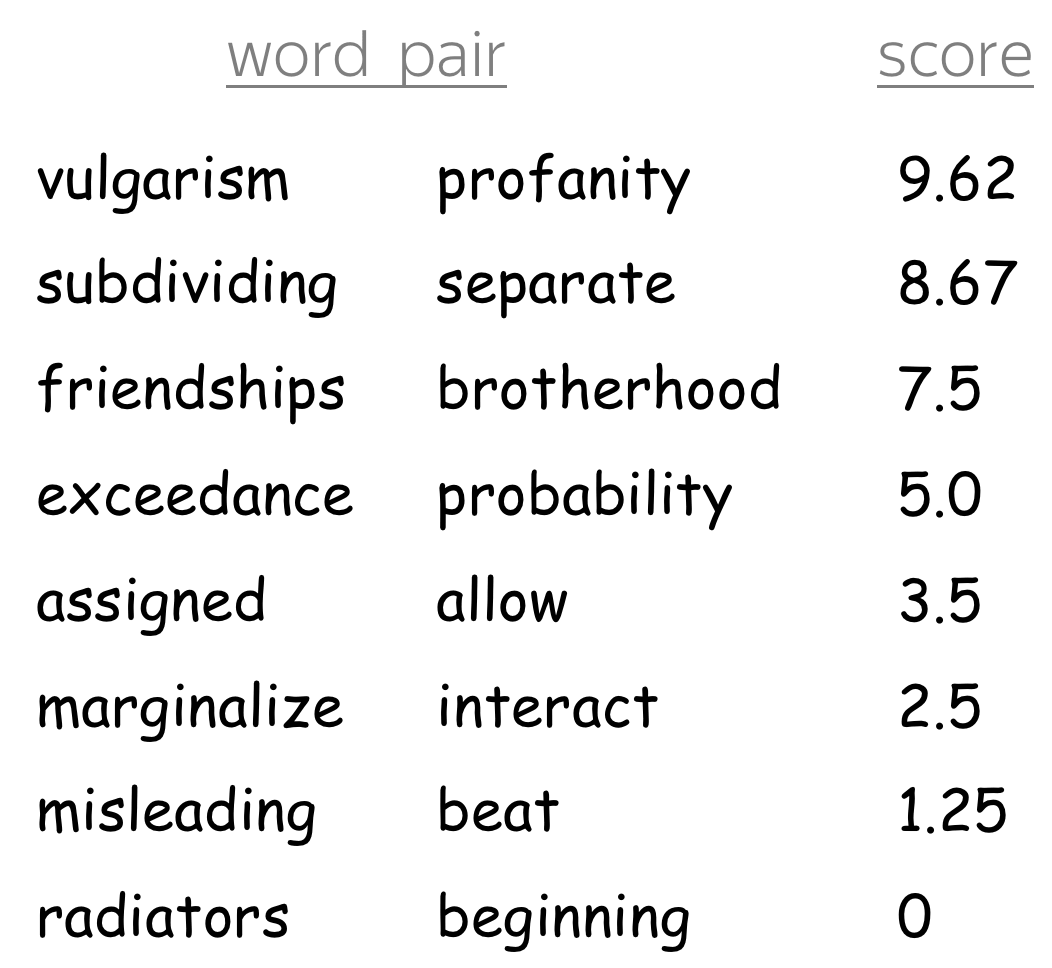Several pairs from the Rare Words similarity benchmark.

### Word Similarity Benchmarks

"Looking" at nearest neighbors (by cosine similarity or Euclidean distance) is one of the methods to estimate the quality of the learned embeddings. There are several word similarity benchmarks (test sets). They consist of word pairs with a similarity score according to human judgments. The quality of embeddings is estimated as the correlation between the two similarity scores (from model and from humans).

## Linear Structure

While similarity results are encouraging, they are not surprising: all in all, the embeddings were trained specifically to reflect word similarity. What is surprising, is that many semantic and syntactic relationships between words are (almost) linear in word vector space.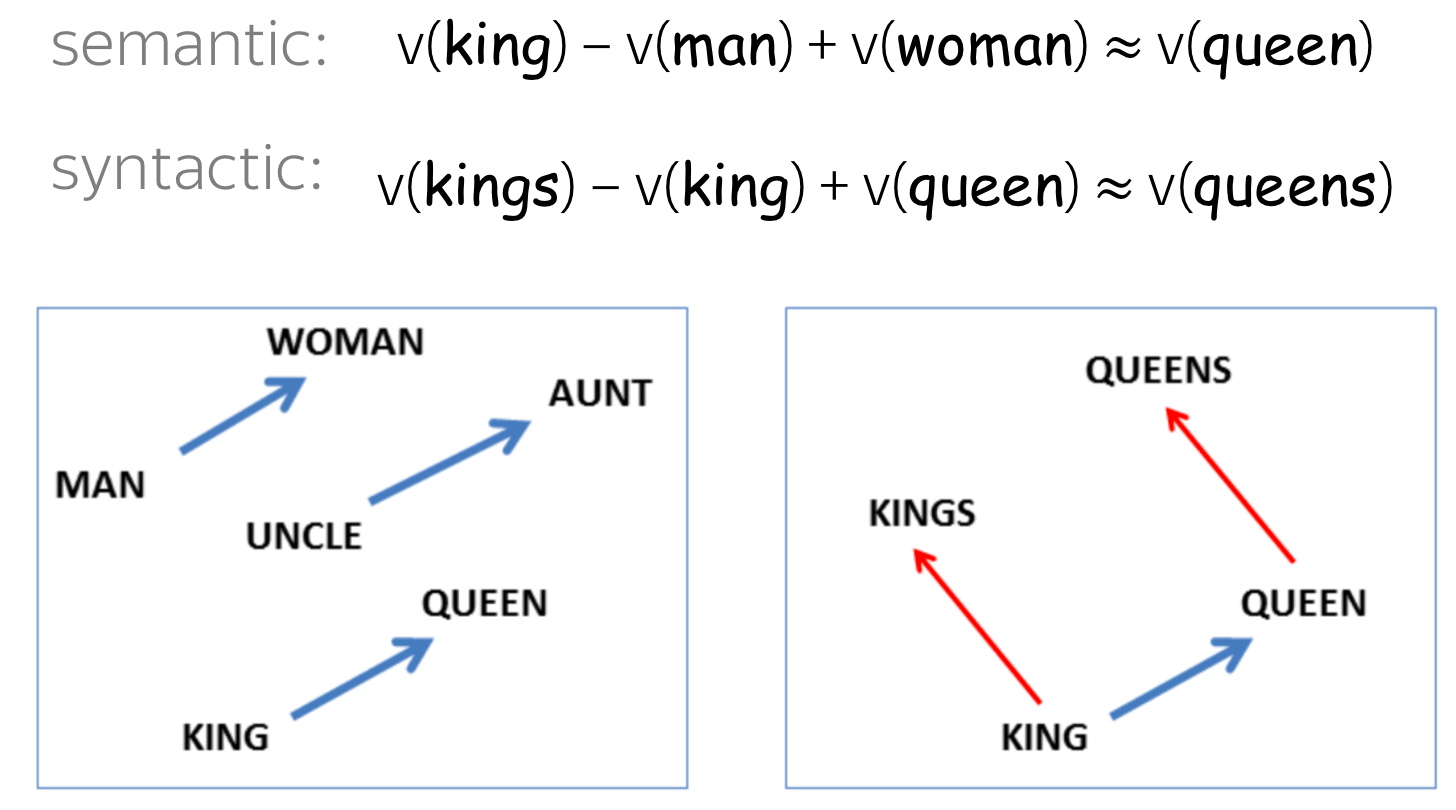For example, the difference between king and queen is (almost) the same as between man and woman. Or a word that is similar to queen in the same sense that kings is similar to king turns out to be queens. The man-woman $$\approx$$ king-queen example is probably the most popular one, but there are also many other relations and funny examples.

Below are examples for the country-capital relation and a couple of syntactic relations.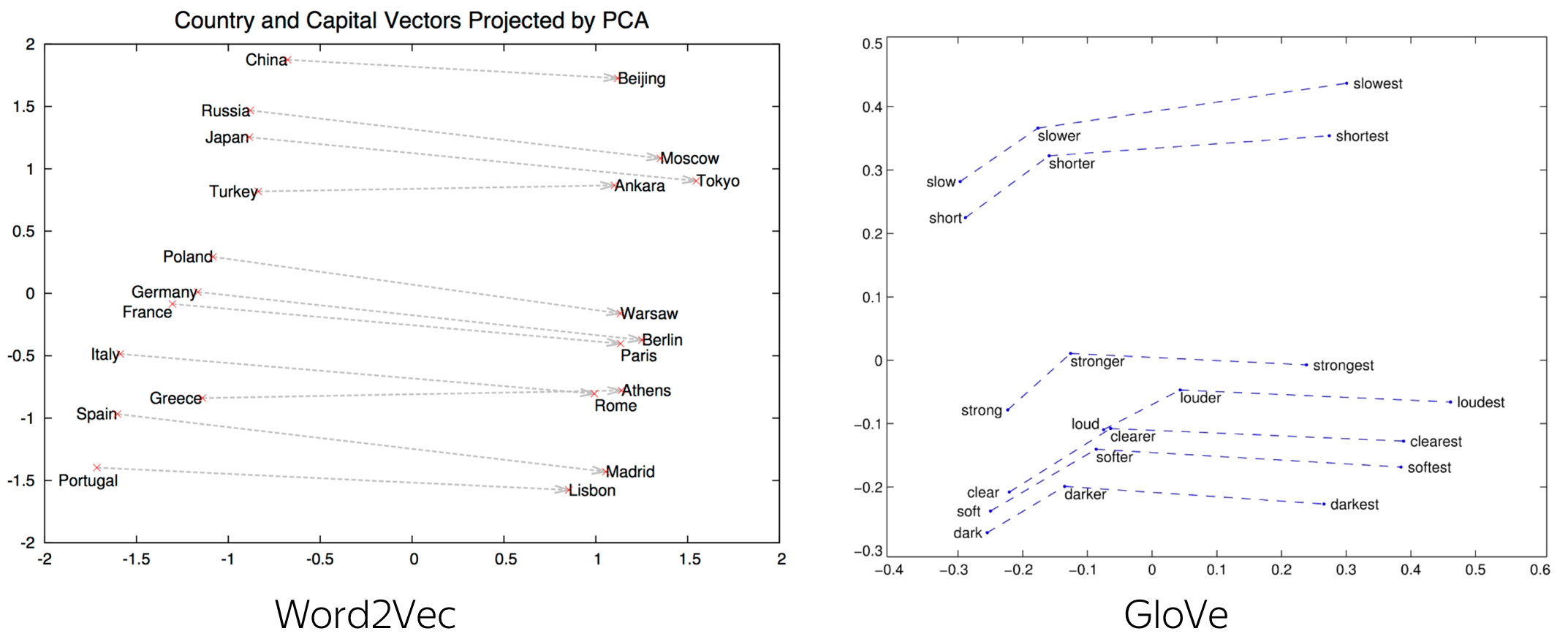At ICML 2019, it was shown that there's actually a theoretical explanation for analogies in Word2Vec. More details are here.

Lena: This paper, Analogies Explained: Towards Understanding Word Embeddings by Carl Allen and Timothy Hospedales from the University of Edinburgh, received Best Paper Honourable Mention award at ICML 2019 - well deserved!

### Word Analogy Benchmarks

These near-linear relationships inspired a new type of evaluation: word analogy evaluation.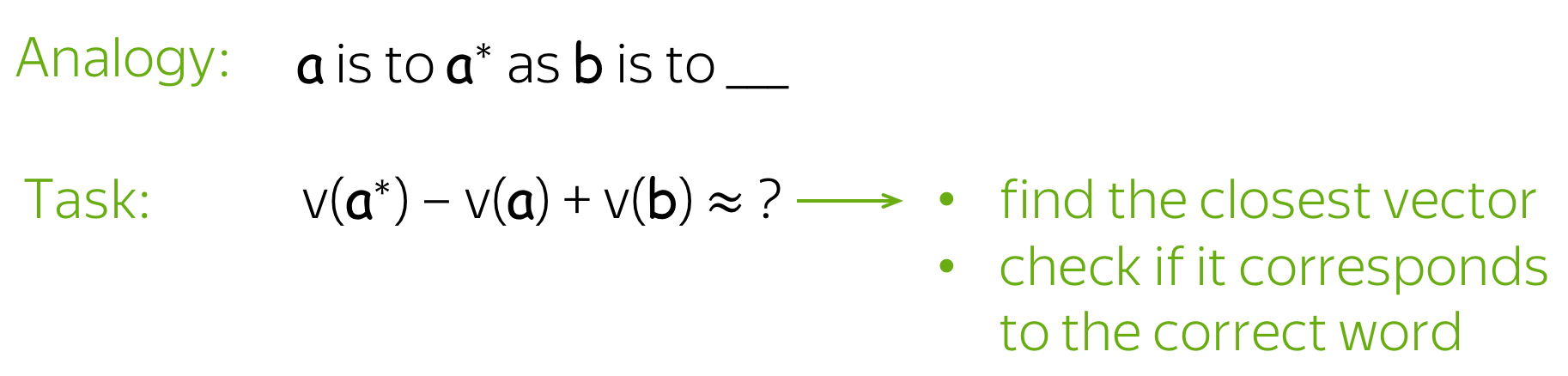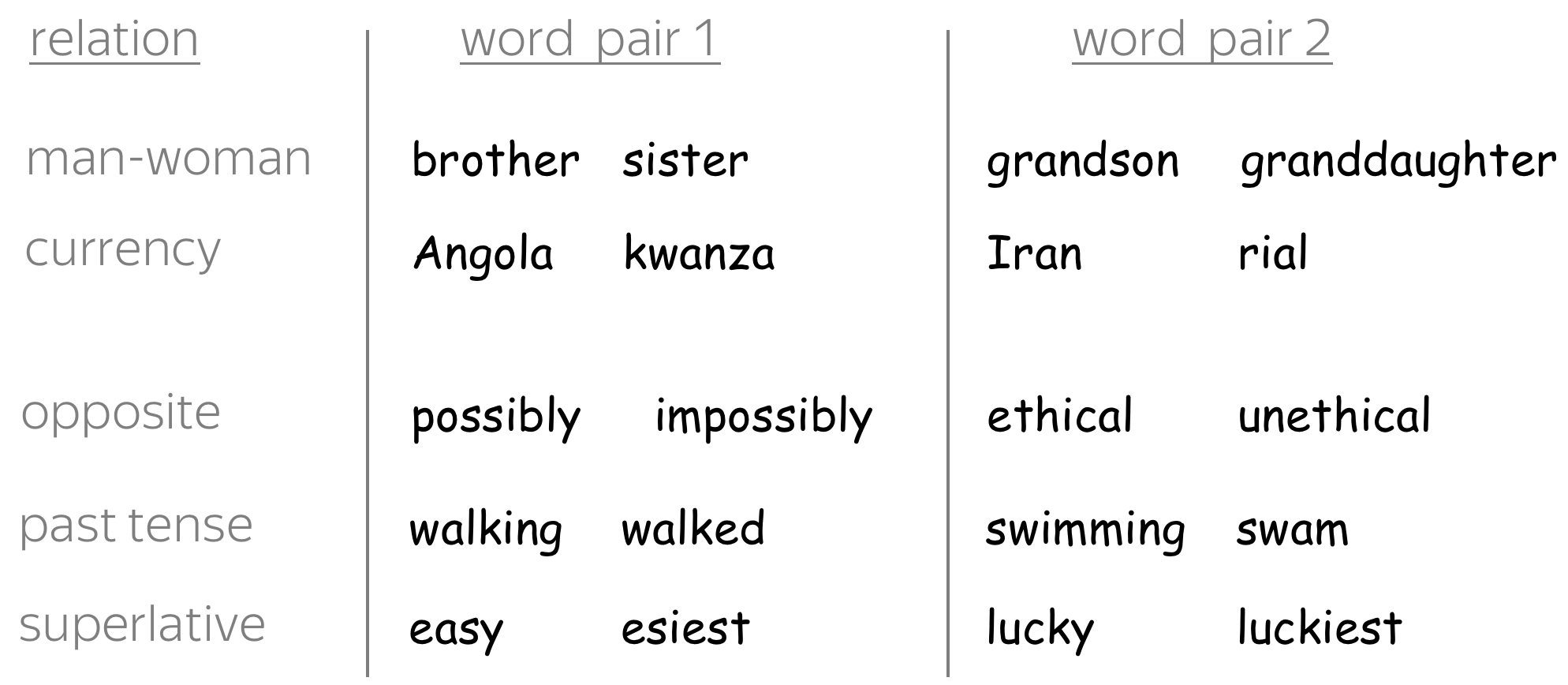Examples of relations and word pairs from the Google analogy test set.

Given two word pairs for the same relation, for example (man, woman) and (king, queen), the task is to check if we can identify one of the words based on the rest of them. Specifically, we have to check if the closest vector to king - man + woman corresponds to the word queen.

Now there are several analogy benchmarks; these include the standard benchmarks (MSR + Google analogy test sets) and BATS (the Bigger Analogy Test Set).

## Similarities across Languages

We just saw that some relationships between words are (almost) linear in the embedding space. But what happens across languages? Turns out, relationships between semantic spaces are also (somewhat) linear: you can linearly map one semantic space to another so that corresponding words in the two languages match in the new, joint semantic space.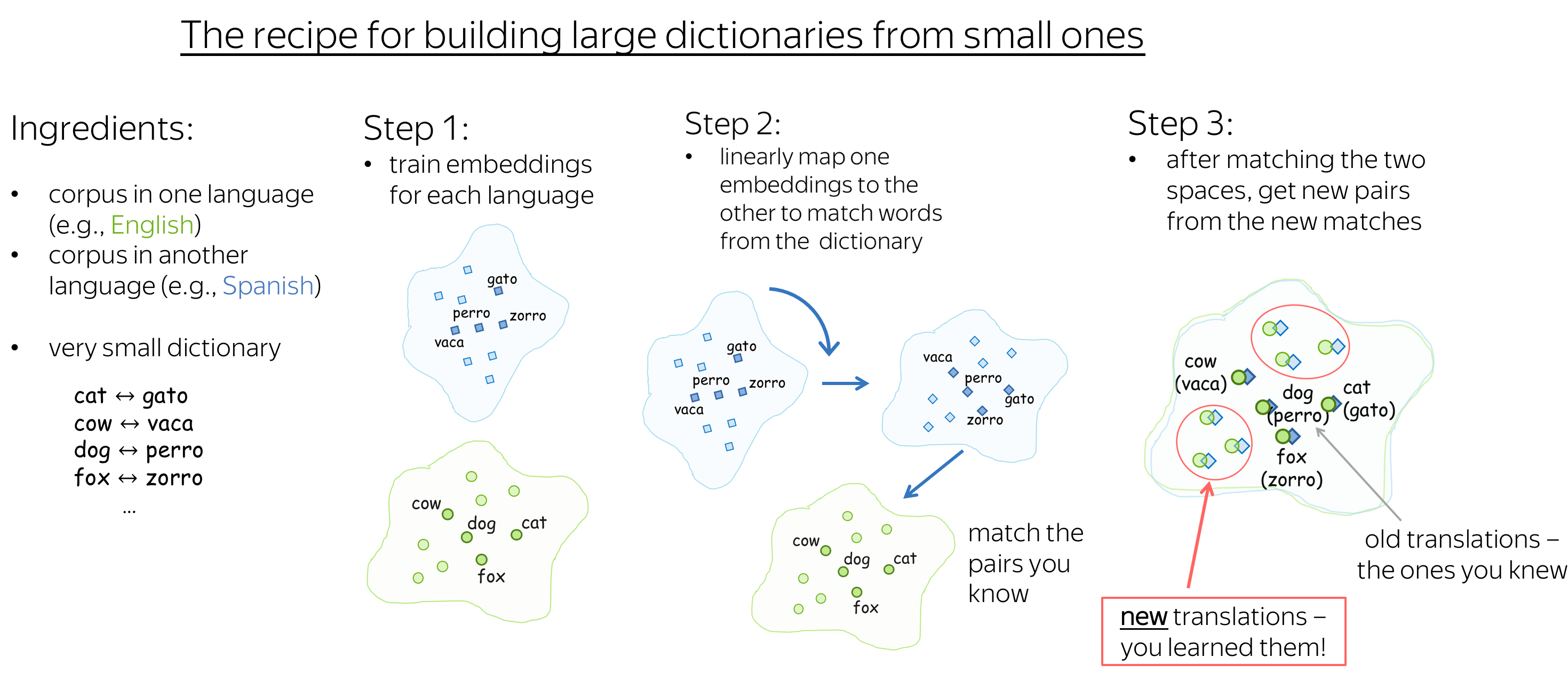The figure above illustrates the approach proposed by Tomas Mikolov et al. in 2013 not long after the original Word2Vec. Formally, we are given a set of word pairs and their vector representations $$\{\color{#88a635}{x_i}\color{black}, \color{#547dbf}{z_i}\color{black} \}_{i=1}^n$$, where $$\color{#88a635}{x_i}$$ and $$\color{#547dbf}{z_i}$$ are vectors for i-th word in the source language and its translation in the target. We want to find a transformation matrix W such that $$W\color{#547dbf}{z_i}$$ approximates $$\color{#88a635}{x_i}$$ : "matches" words from the dictionary. We pick $$W$$ such that $W = \arg \min\limits_{W}\sum\limits_{i=1}^n\parallel W\color{#547dbf}{z_i}\color{black} - \color{#88a635}{x_i}\color{black}\parallel^2,$ and learn this matrix by gradient descent.

In the original paper, the initial vocabulary consists of the 5k most frequent words with their translations, and the rest is learned.Later it turned out, that we don't need a dictionary at all - we can build a mapping between semantic spaces even if we know nothing about languages! More details are here.Is the "true" mapping between languages indeed linear, or more complicated? We can look at geometry of the learned semantic spaces and check. More details are here.The idea to linearly map different embedding sets to (nearly) match them can also be used for a very different task! Learn more in the Research Thinking section.# Research Thinking

How to

• Read the short description at the beginning - this is our starting point, something known.
• Read a question and think: for a minute, a day, a week, ... - give yourself some time! Even if you are not thinking about it constantly, something can still come to mind.
• Look at the possible answers - previous attempts to answer/solve this problem.
Important: You are not supposed to come up with something exactly like here - remember, each paper usually takes the authors several months of work. It's a habit of thinking about these things that counts! All the rest a scientist needs is time: to try-fail-think until it works.

It's well-known that you will learn something easier if you are not just given the answer right away, but if you think about it first. Even if you don't want to be a researcher, this is still a good way to learn things!

## Count-Based Methods

Improve Simple Co-Occurrence Counts

The simplest co-occurrence counts treat context words equally, although these words are at different relative positions from the central word. For example, from one sentence the central word cat will get a co-occurrence count of 1 for each of the words cute, grey, playing, in (look at the example to the right).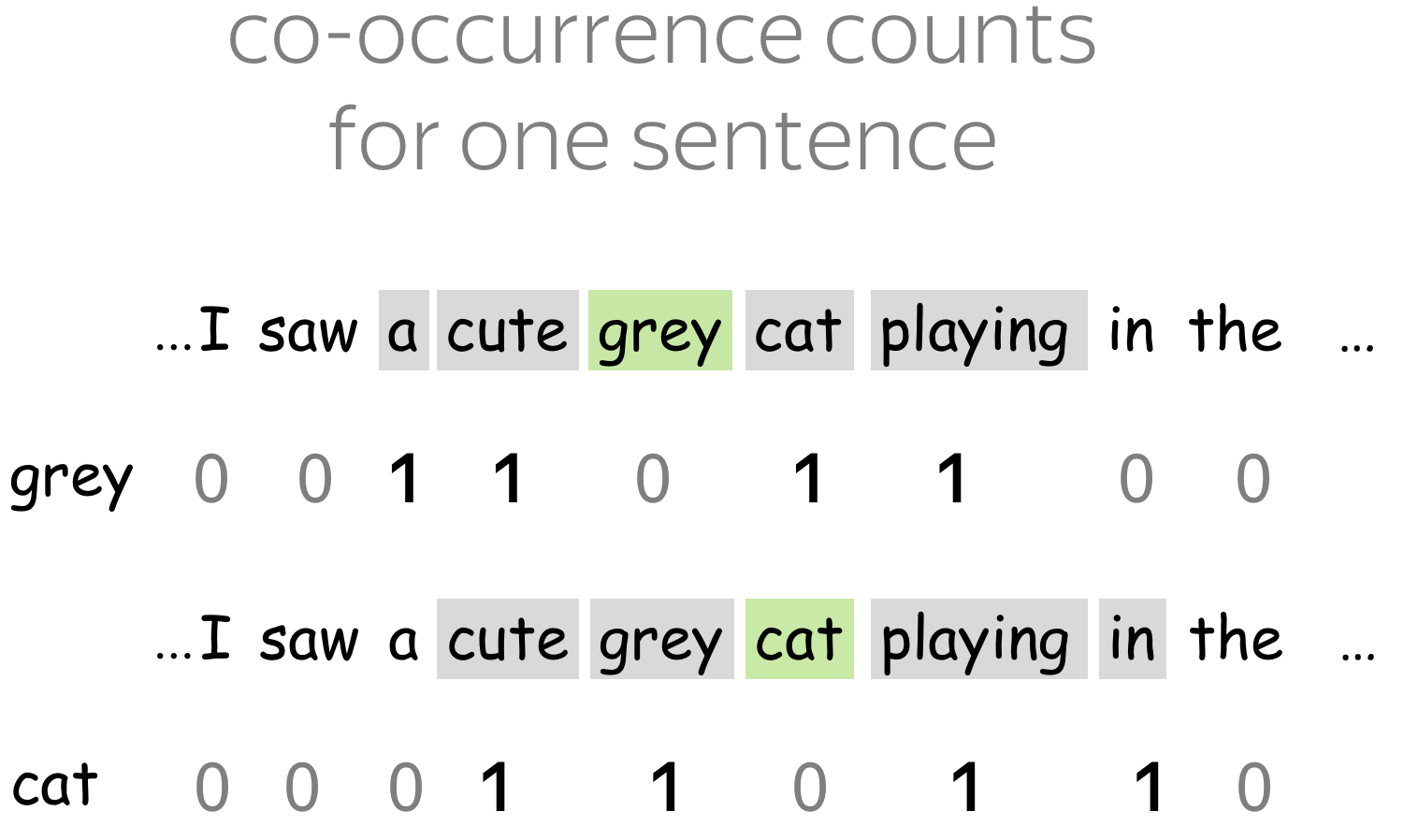? Are context words at different distances equally important? If not, how can we modify co-occurrence counts?
Possible answers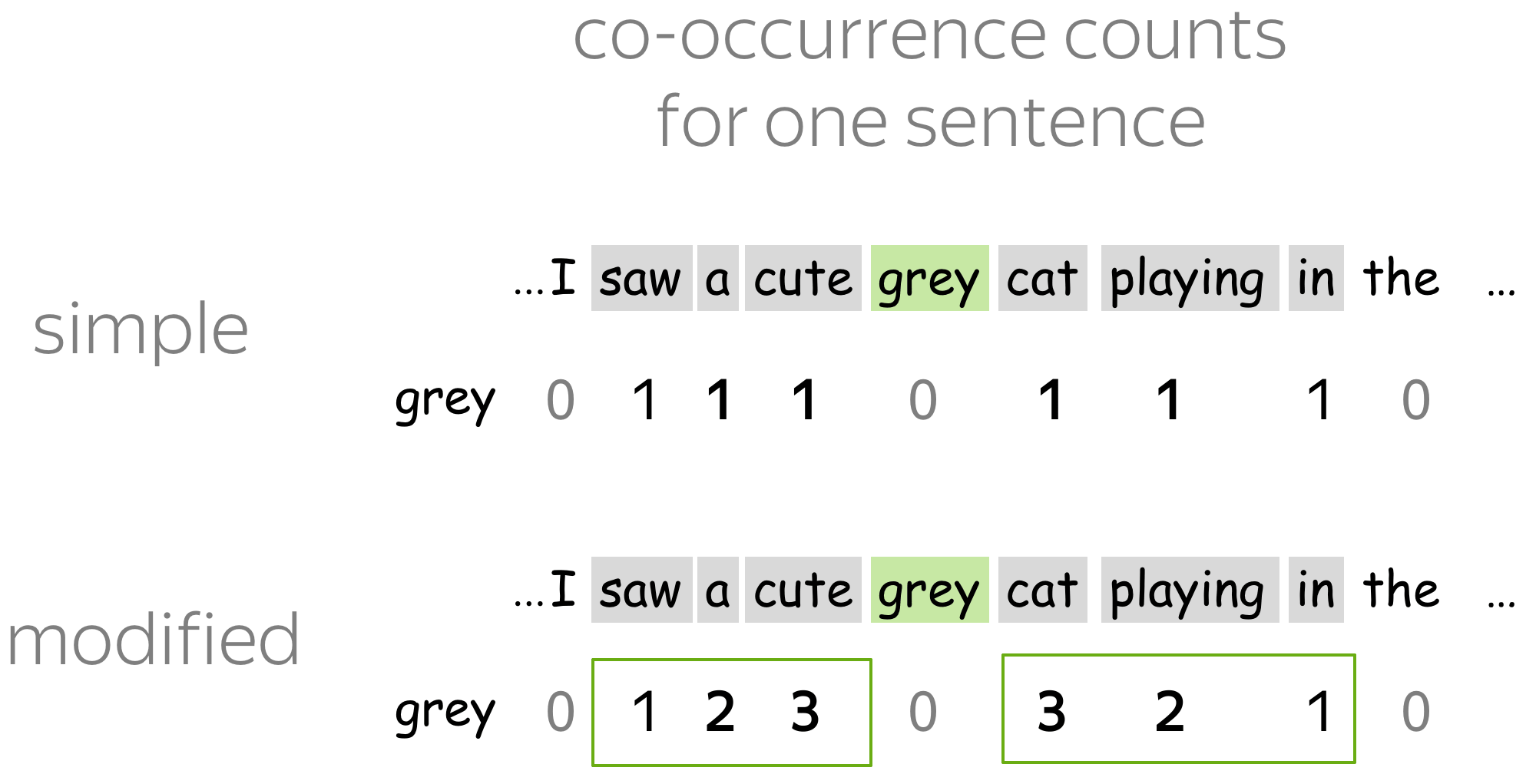Intuitively, words that are closer to the central are more important; for example, immediate neighbors are more informative than words at distance 3.

We can use this to modify the model: when evaluating counts, let's give closer words more weight. This idea was used in the HAL model (1996), which once was very famous. They modified counts as shown in the example.

? In language, word order is important; specifically, left and right contexts have different meanings. How can we distinguish between the left and right contexts?
One of the existing approaches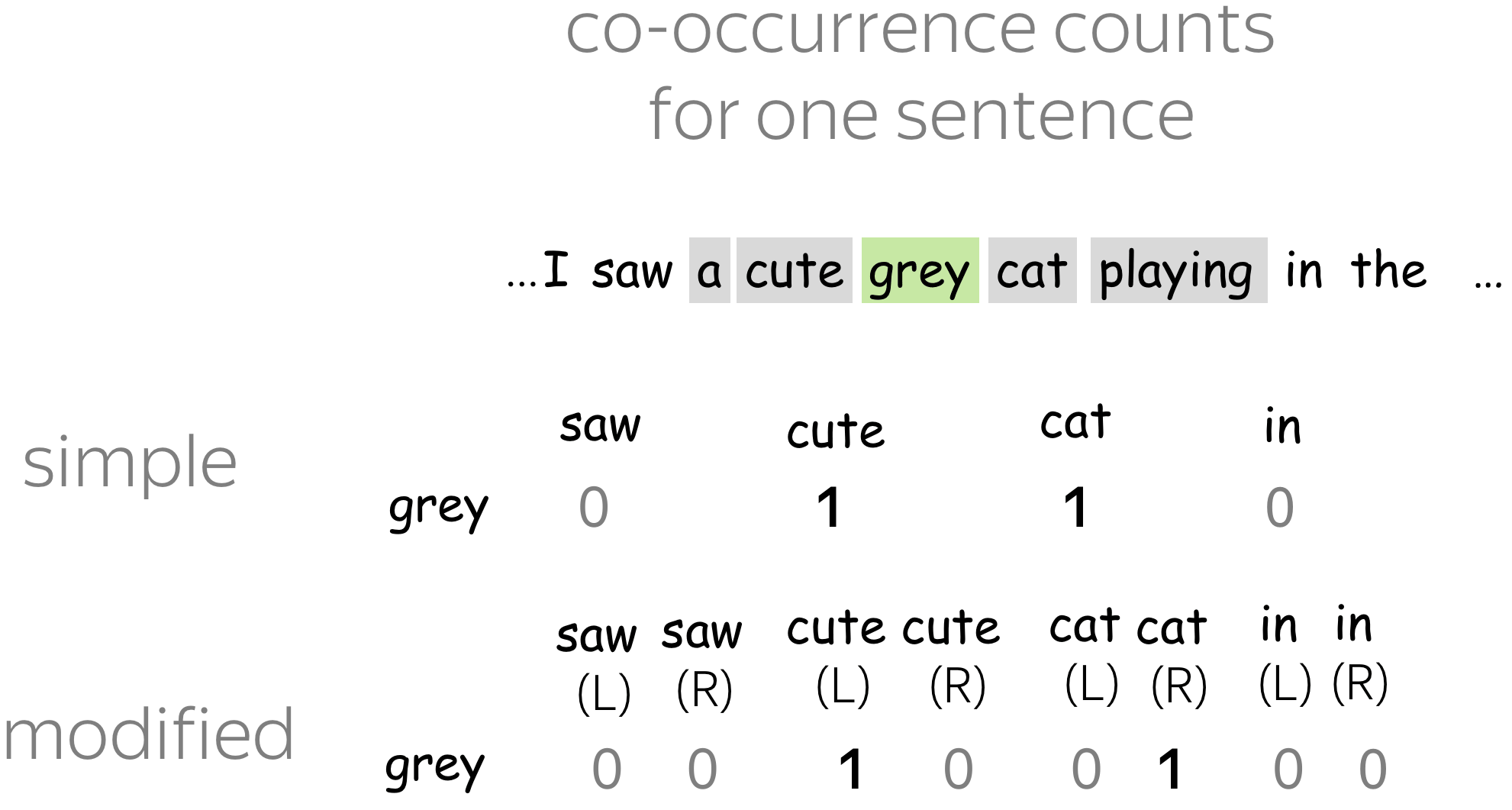Here the weighting idea we saw above would not work: we can not say which contexts, left or right, are more important.

What we have to do is to evaluate co-occurrences to the left and to the right separately. For each context word, we will have two different counts: one when it is a left context and another when it is the right context. This means that our co-occurrence matrix will have |V| rows and 2|V| columns. This idea was also used in the HAL model (1996).

Look at the example; note that for cute, we have left co-occurrence count, for cat - right.

## Word2Vec

Are all context words equally important for training?

During Word2Vec training, we make an update for each of the context words. For example, for the central word cat we make an update for each of the words cute, grey, playing, in.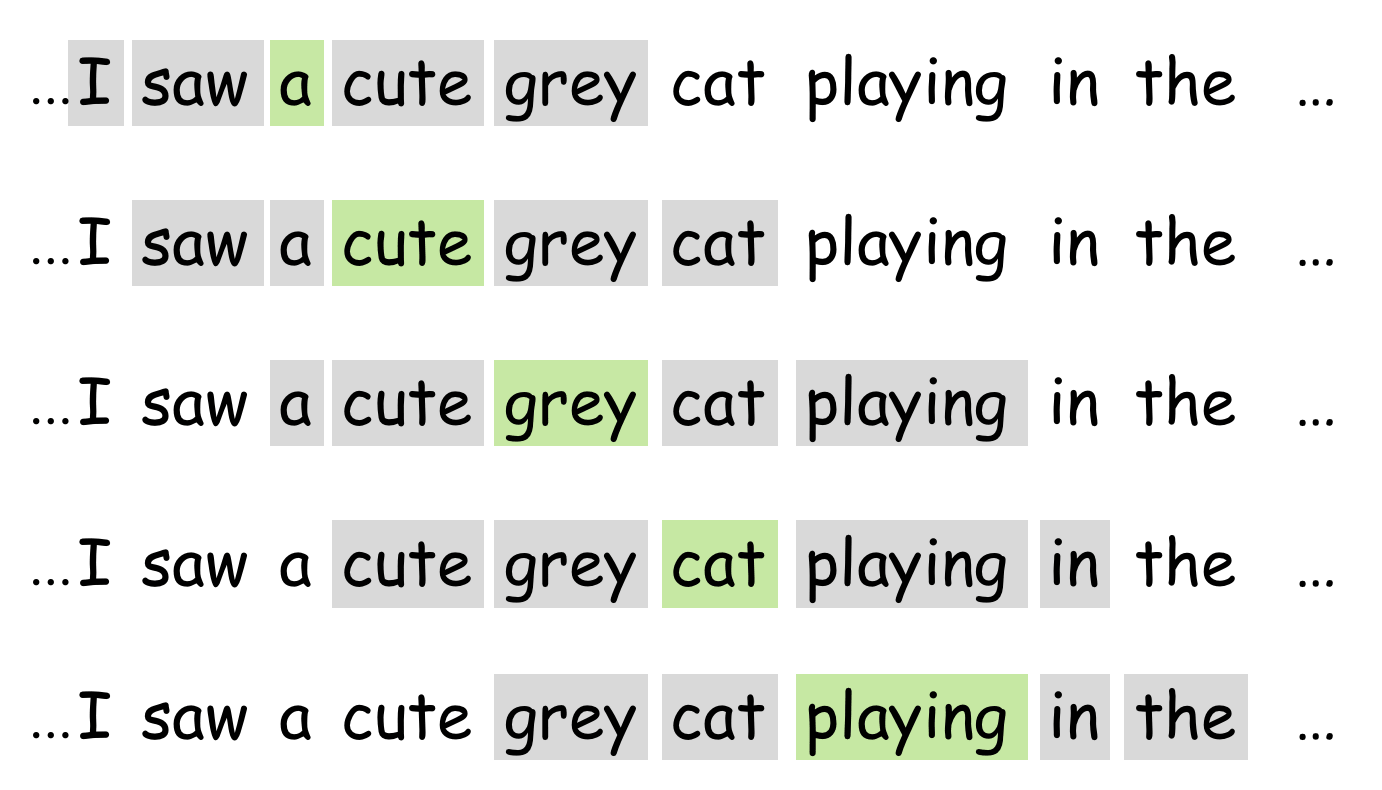? Are all context words equally important?
Which word types give more/less information than others? Think about some characteristics of words that can influence their importance. Do not forget the previous exercise!
• word frequency
We can expect that frequent words usually give less information than rare ones. For example, the fact that cat appears in context of in does not tell us much about the meaning of cat: the word in serves as a context for many other words. In contrast, cute, grey and playing already give us some idea about cat.
• distance from the central word
As we discussed in the previous exercise on count-based methods, words that are closer to the central may be more important.

? How can we use this to modify training?
Tricks from the original Word2Vec

### 1. Word Frequency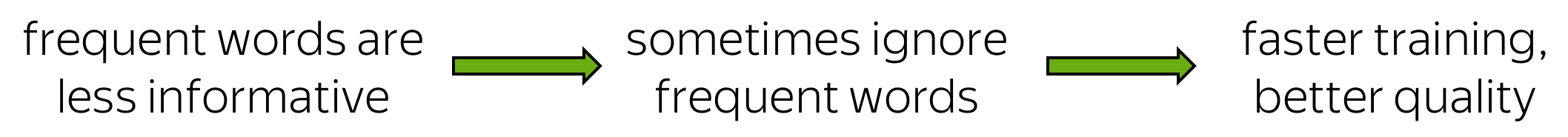To account for different informativeness of rare and frequent words, Word2Vec uses a simple subsampling approach: each word $$w_i$$ in the training set is ignored with probability computed by the formula $P(w_i)=1 - \sqrt{\frac{thr}{f(w_i)}}$ where $$f(w_i)$$ is the word frequency and $$thr$$ is the chosen threshold (in the original paper, $$thr=10^{-5}$$). This formula preserves the ranking of the frequencies, but aggressively subsamples words whose frequency is greater than $$thr$$.

Interestingly, this heuristic works well in practice: it accelerates learning and even significantly improves the accuracy of the learned vectors of the rare words.

### 2. Distance from the central word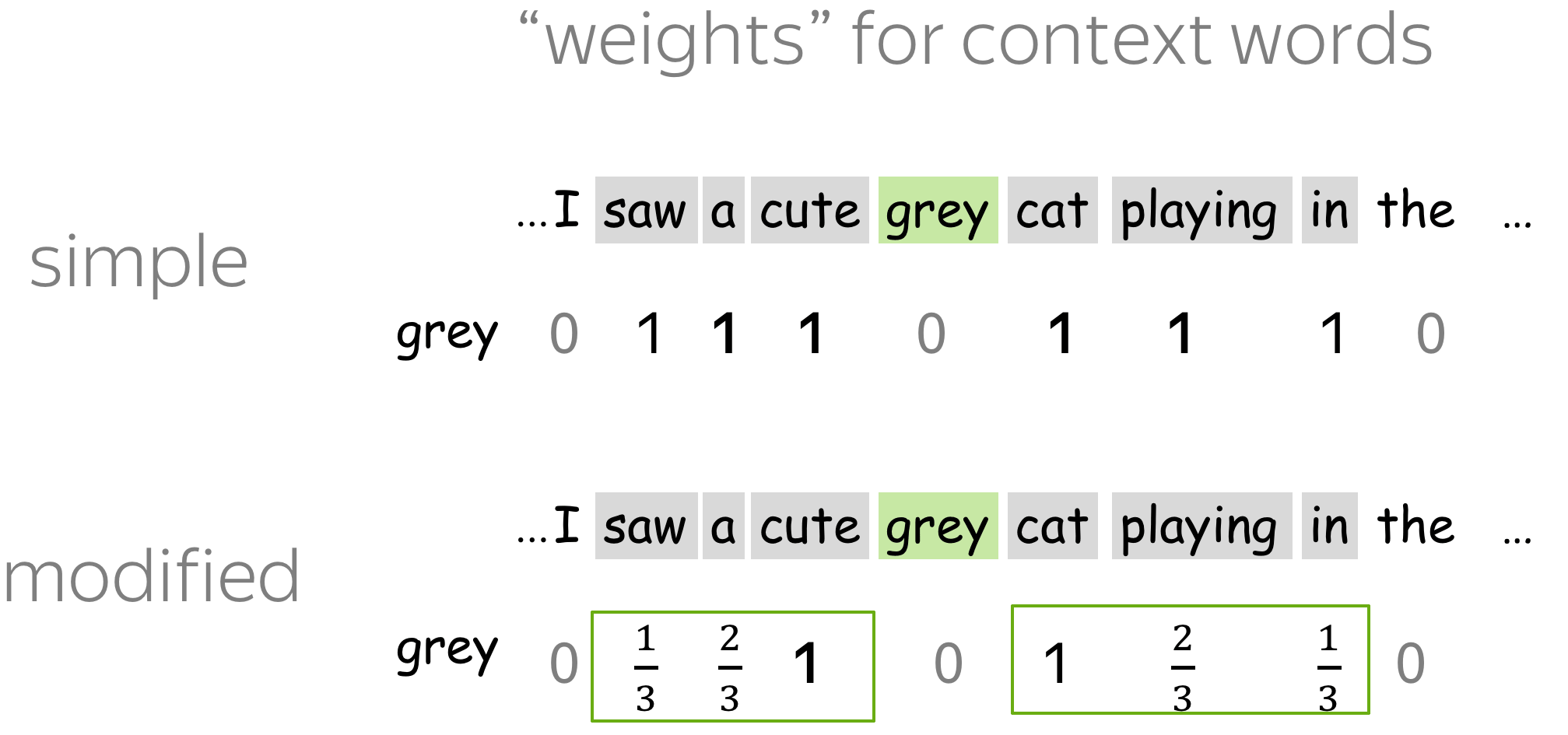As in the previous exercise on count-based methods, we can assign higher weights to the words which are closer to the central.

At the first glance, you won't see any weights in the original Word2Vec implementation. However, at each step it samples the size of the context window from 1 to L. Therefore, words which are closer to central are used more frequently than the distant ones. In the original work this was (probably) done for efficiency (fewer updates for each step), but this also has the effect similar to assigning weights.
Use Information About Subwords ("invent" FastText)

Usually, we have a look-up table where each word is assigned a distinct vector. By construction, these vectors do not have any idea about subwords they consist of: all information they have is what they learned from contexts.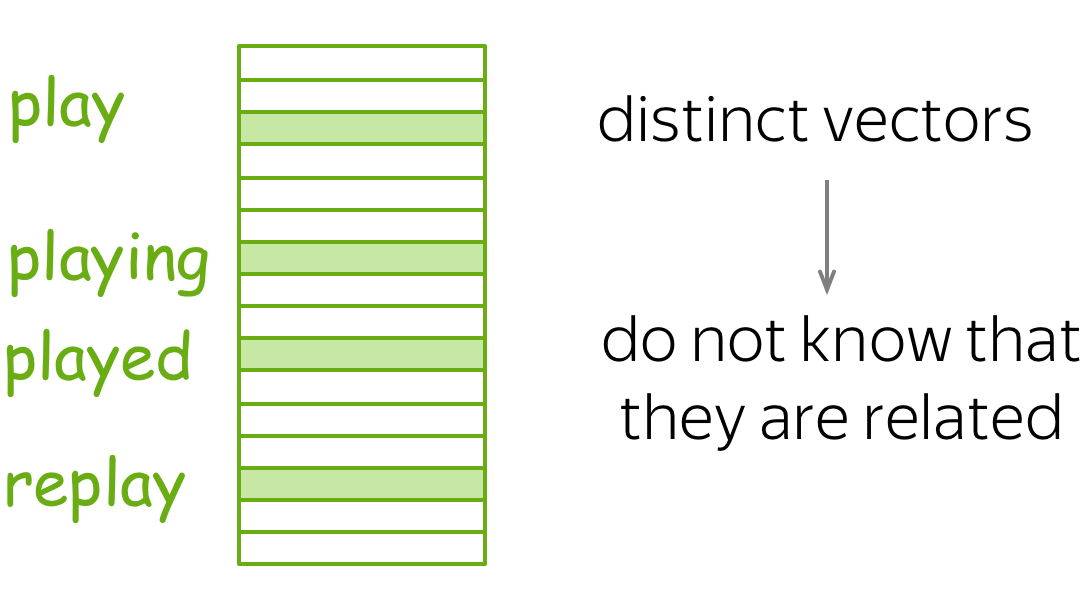? Imagine that word embeddings have some understanding of subwords they consist of. Why can this be useful?
• better understanding of morphology
By assigning a distinct vector to each word, we ignore morphology. Giving information about subwords can let the model know that different tokens can be forms of the same word.
• representations for unknown words
Usually, we can represent only those words, which are present in the vocabulary. Giving information about subwords can help to represent out-of-vocabulary words relying of their spelling.
• handling misspellings
Even if one character in a word is wrong, this is another token, and, therefore, a completely different embedding (or even unknown word). With information about subwords, misspelled word would still be similar to the original one.

? How can we incorporate information about subwords into embeddings? Let's assume that the training pipeline is fixed, e.g., Skip-Gram with Negative sampling.
One of the existing approaches (FastText)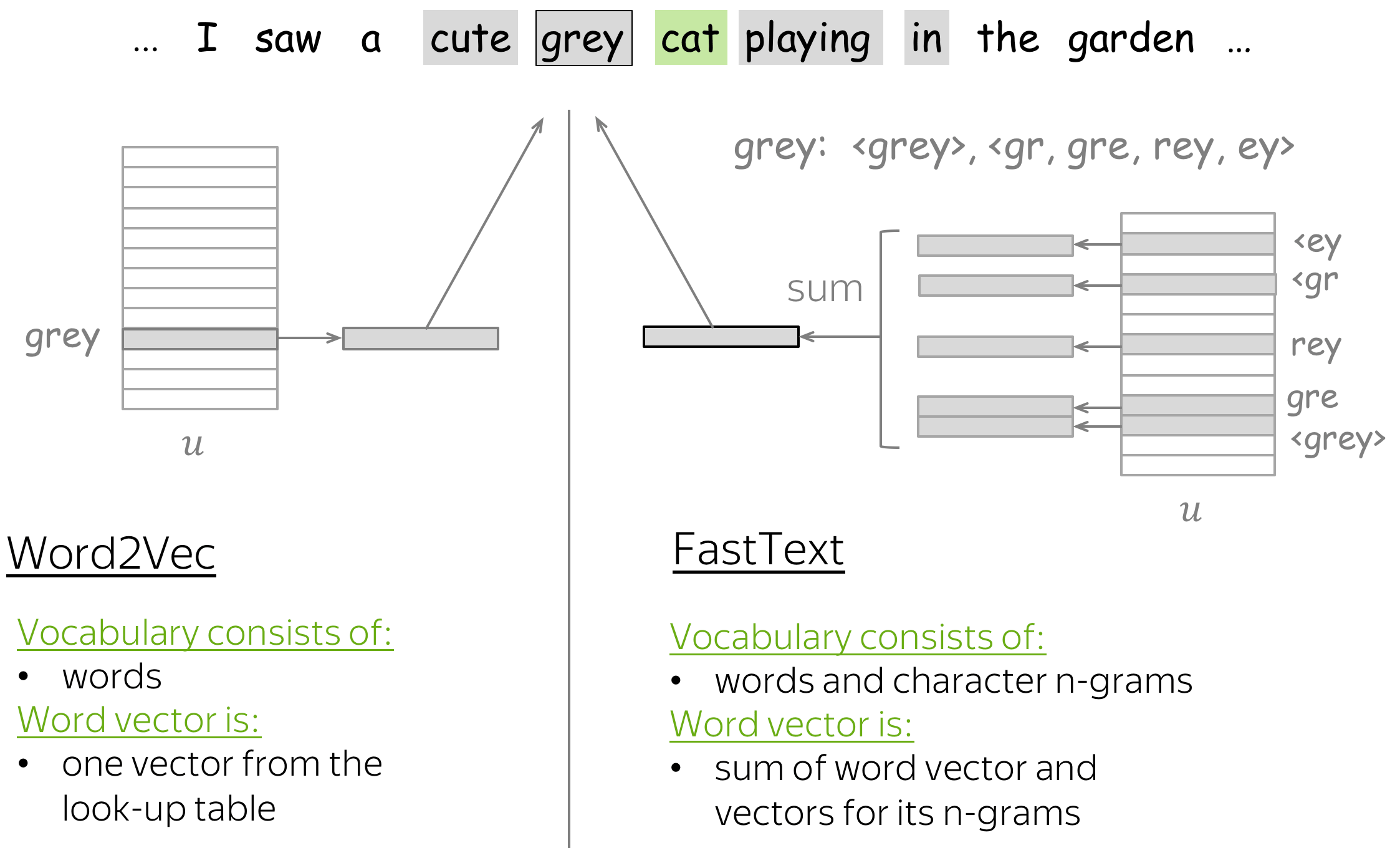One of the possible approaches is to compose a word vector from vectors for its subwords. For example, popular FastText embeddings operate as shown in the illustration. For each word, they add special start and end characters for each word. Then, in addition to the vector for this word, they also use vectors for character n-grams (which are also in the vocabulary). Representation of a word us sum of vectors for the word and its subwords, as shown in the picture.

Note that this changes only the way we form word vector; the whole training pipeline is the same as in the standard Word2Vec.

## Semantic Change

Detect Words that Changed Their Usage

Imagine you have text corpora from different sources: time periods, populations, geographic regions, etc. In digital humanities and computational social science, people often want to find words that used differently in these corpora.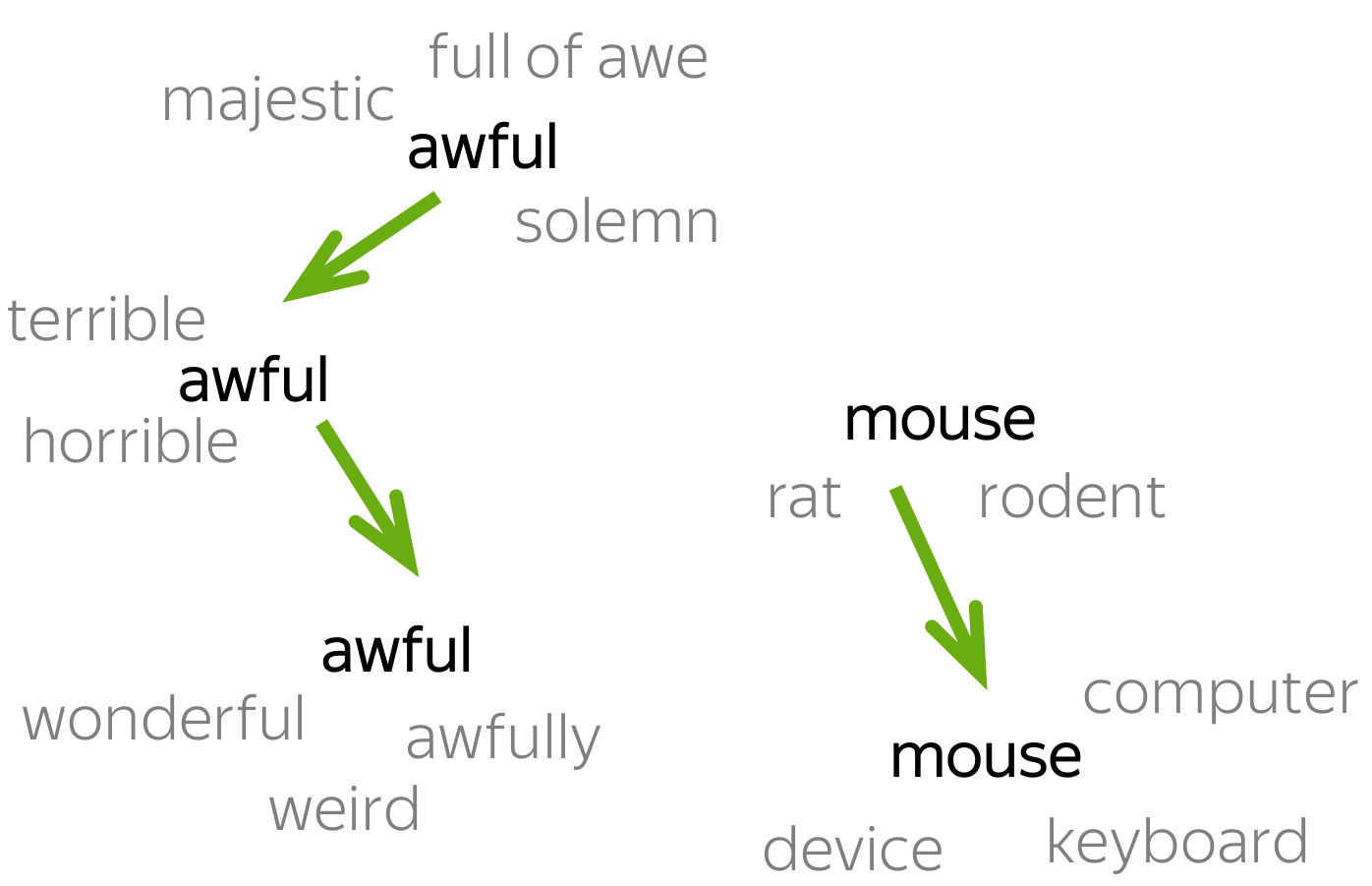? Given two text corpora, how would you detect which words are used differently/have different meaning? Do not be shy to think about very simple ways!
Some of the existing attempts

### ACL 2020: train embeddings, look at the neighbors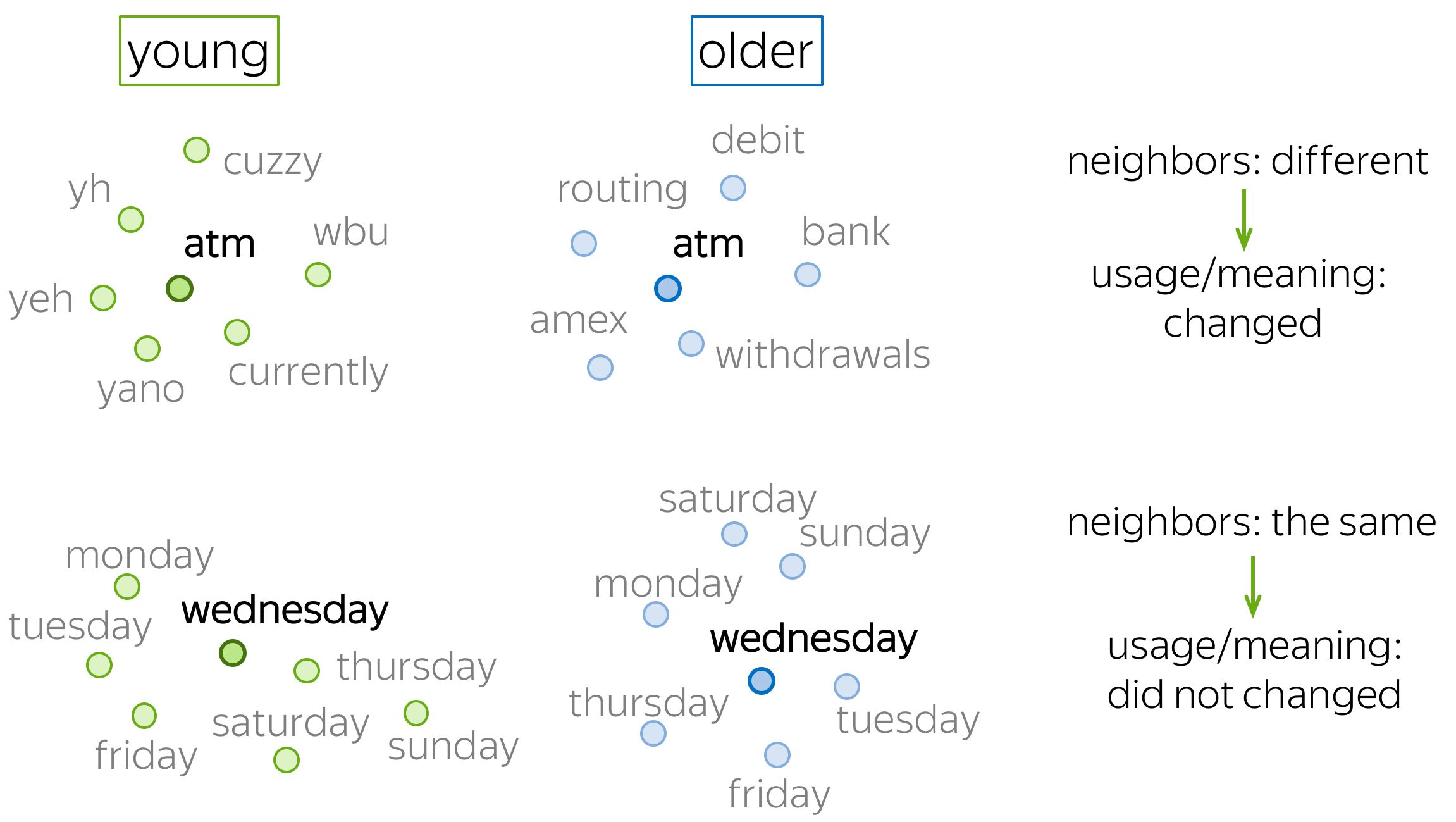A very simple approach is to train embeddings (e.g., Word2Vec) and look at the closest neighbors. If a word's closest neighbors are different for the two corpora, the word changed its meaning: remember that word embeddings reflect contexts they saw!

This approach was proposed in this ACL 2020 paper. Formally, for each word the authors take k nearest neighbors in the two embeddings sets and count how many neighbors are the same. Large intersection means that the meaning is not different, small intersection - meaning is different.

Lena: Note that while the approach is recent, it is extremely simple and works better than previous more complicated ideas. Never be afraid to try simple things - you'll be surprised how often they work!

### Previous popular approach: align two embedding sets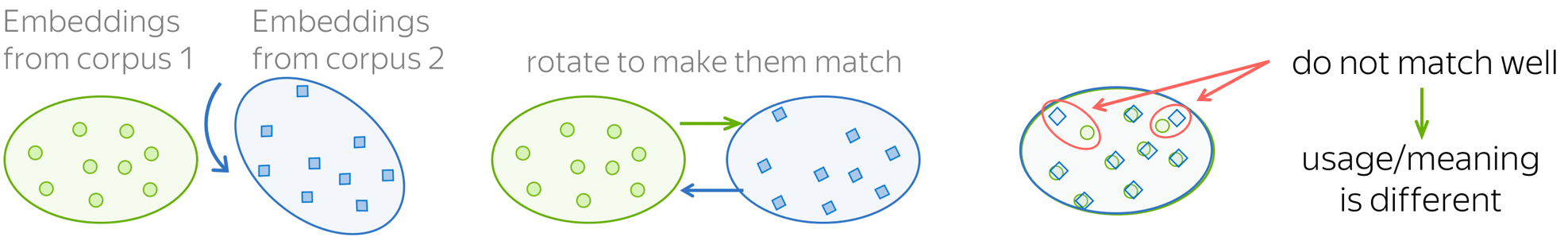The previous popular approach was to align two embeddings sets and to find word whose embeddings do not match well. Formally, let $$\color{#88a635}{W_1}\color{black}, \color{#547dbf}{W_2}\color{black} \in \mathbb{R}^{d\times |V|}$$ be embedding sets trained on different corpora. To align the learned embeddings, the authors find the rotation $$R = \arg \min\limits_{Q^TQ=I}\parallel \color{#547dbf}{W_2}\color{black}Q - \color{#88a635}{W_1}\color{black}\parallel_F$$ - this is called Orthogonal Procrustes. Using this rotation, we can align embedding sets and find words which do not match well: these are the words that change meaning with the corpora.

Lena: You will implement Ortogonal Proctustes in your homework to align Russian and Ukranian embeddings. Find the notebook in the course repo.# Have Fun!

Semantic Space Surfer

Usually, we want word embeddings to reason as humans do. But let's try the opposite: you will try to think as word embeddings.

You will see the analogical example, e.g. king - man + woman = ?, and several possible answers. The task is to guess what word embeddings think.

Complete the task (10 examples) and get a Semantic Space Surfer Certificate!

Word embeddings: we used glove-twitter-100 from gensim-data.

Big thanks Just Heuristic for the help with technical issues! Just Heuristic - Just Fun!